## Friday, January 04, 2019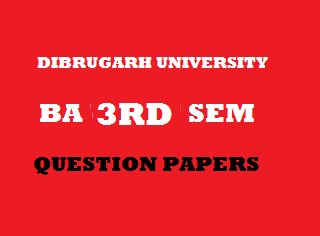2014
(November)
MATHEMATICS
(General)
Course: 301
[(Group – A: Coordinate Geometry and
Group – B: Analysis – I (Real Analysis)]
Full Marks: 80
Pass Marks: 32
Time: 3 hours
The figures in the margin indicate full marks for the questions
GROUP – A
(Coordinate Geometry)
SECTION – I
(2-Dimension)
1. (a) Write what should be done to remove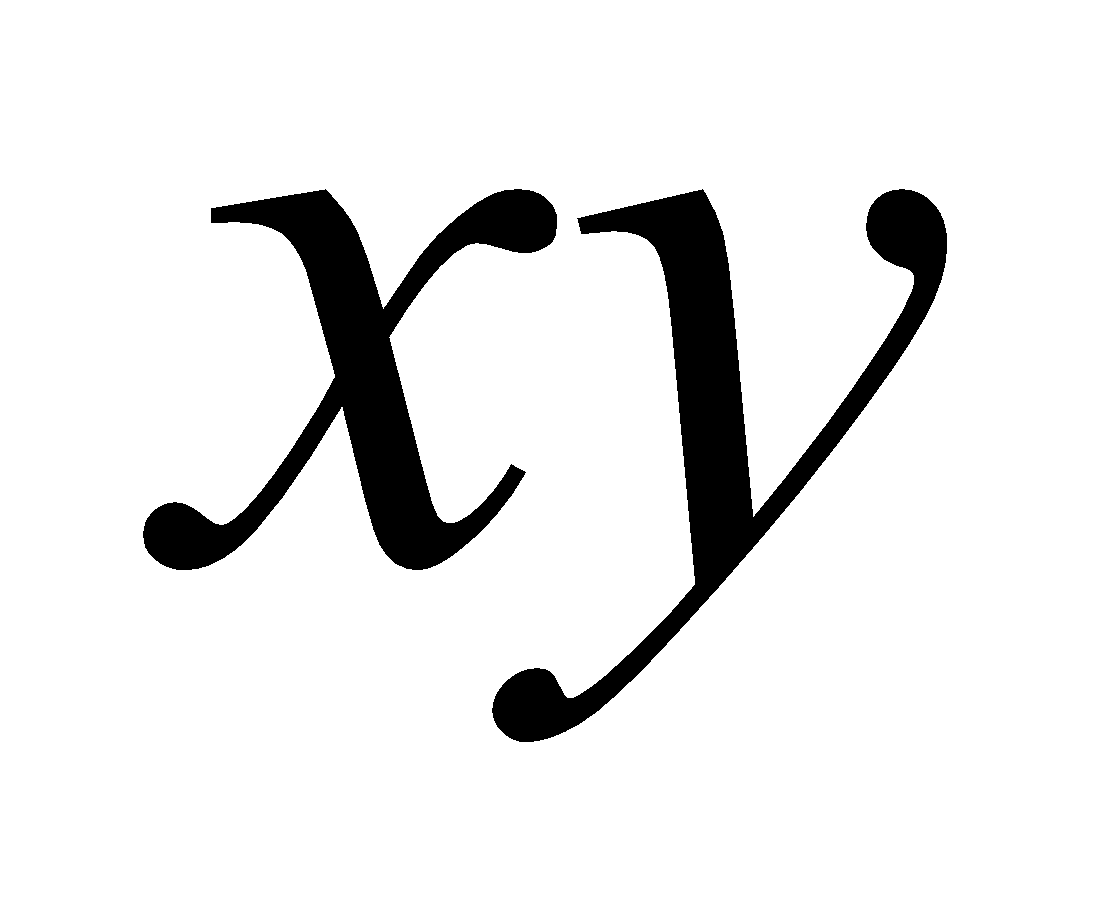term from the equation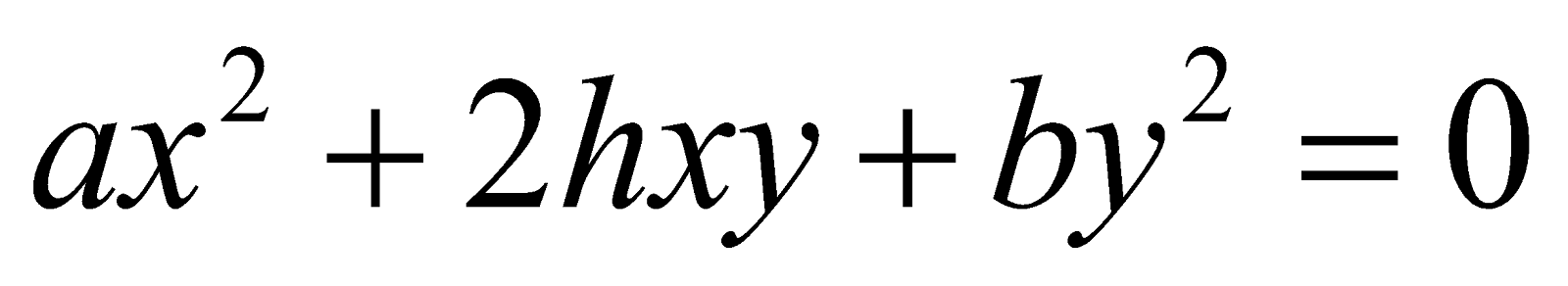. 1
(b) Write the transformed equation of the straight line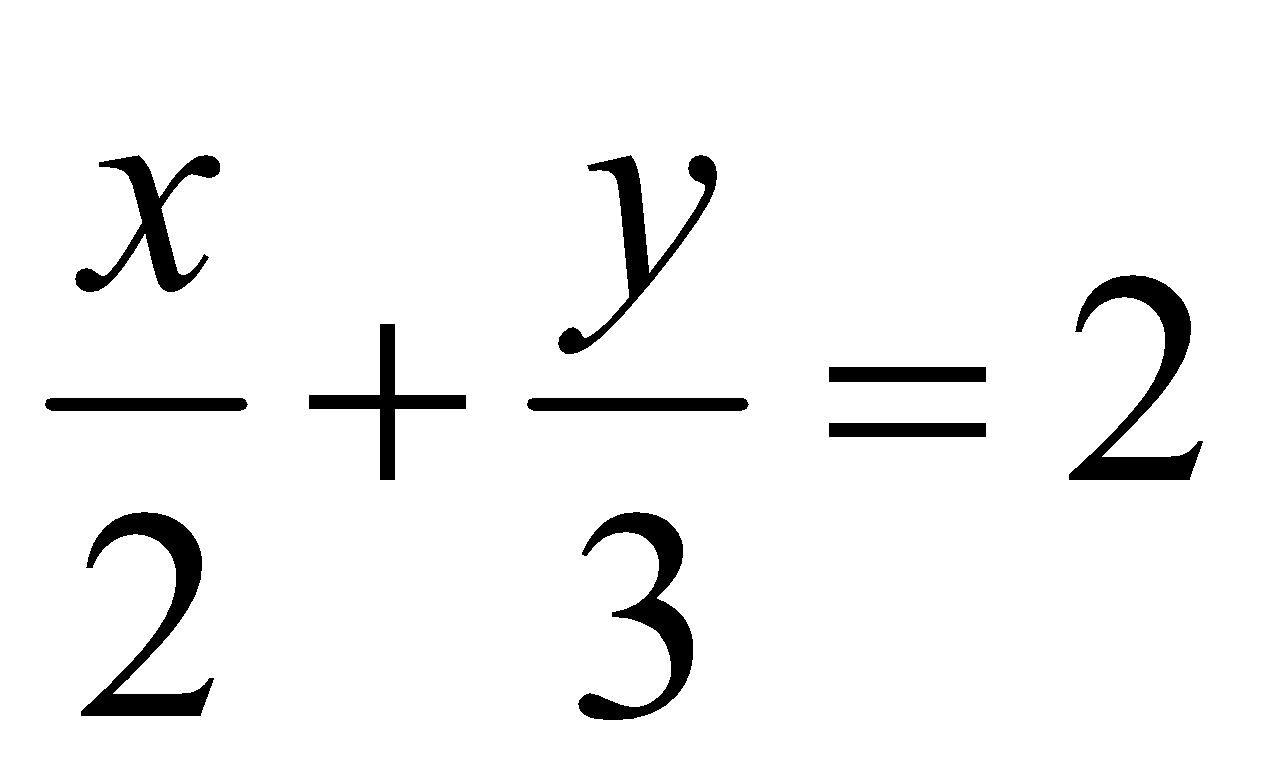when the origin is transformed to the point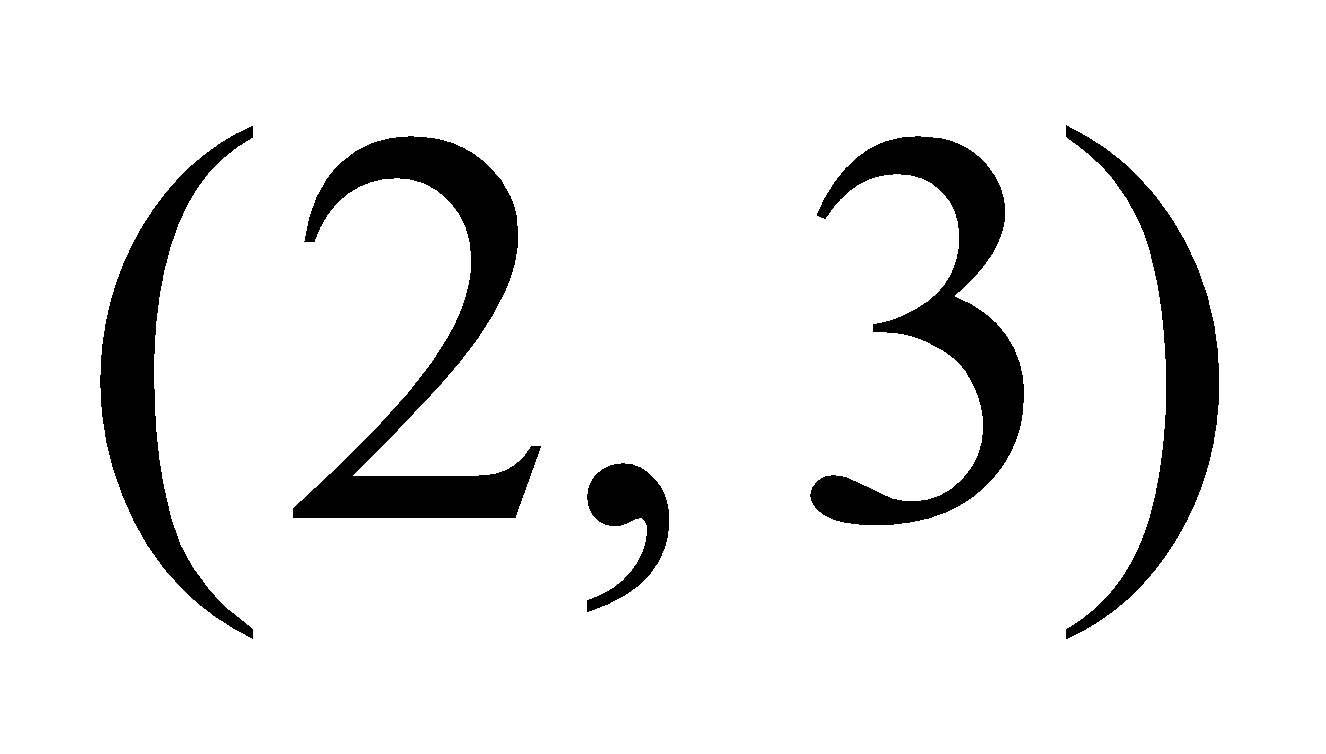.
(c) Find the angle through which the axes must be turned to remove theterm from the equation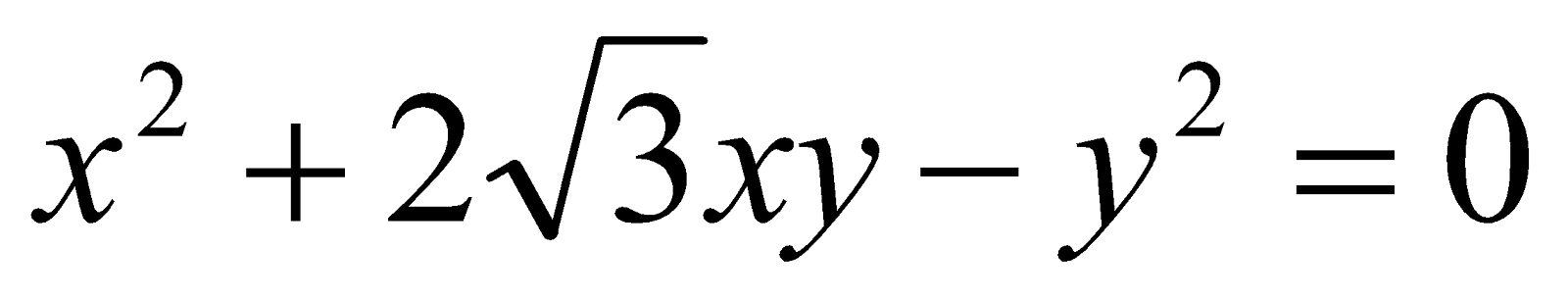.
2. (a) Straight lines represented by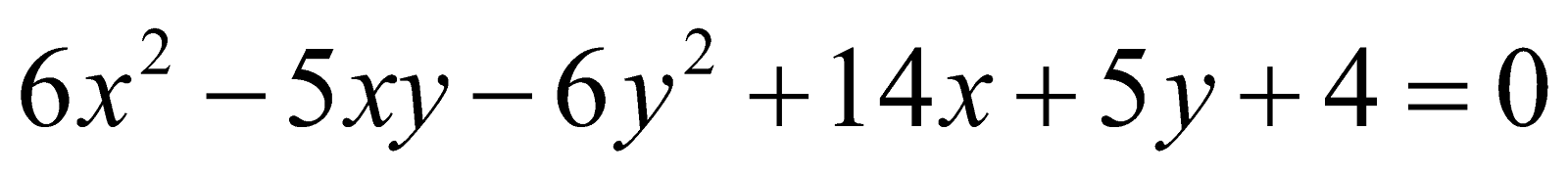pass through the origin. State true or false.
(b) Show that straight lines represented by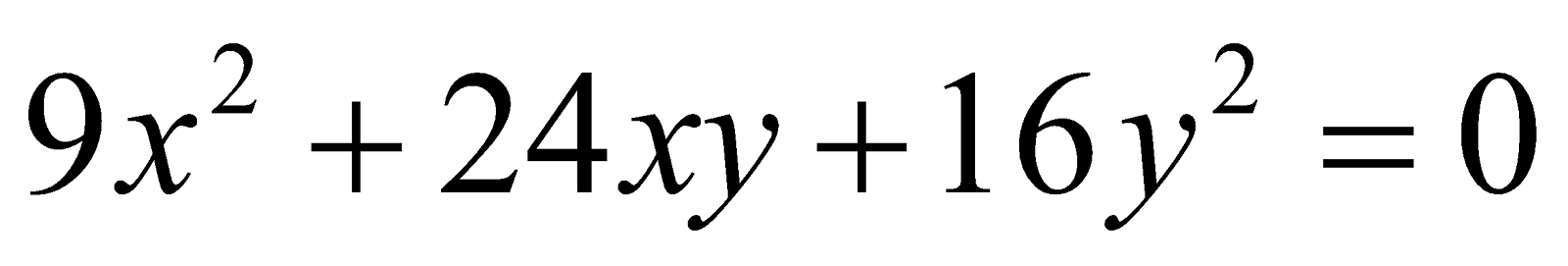are parallel.
(c) Show that the equation 4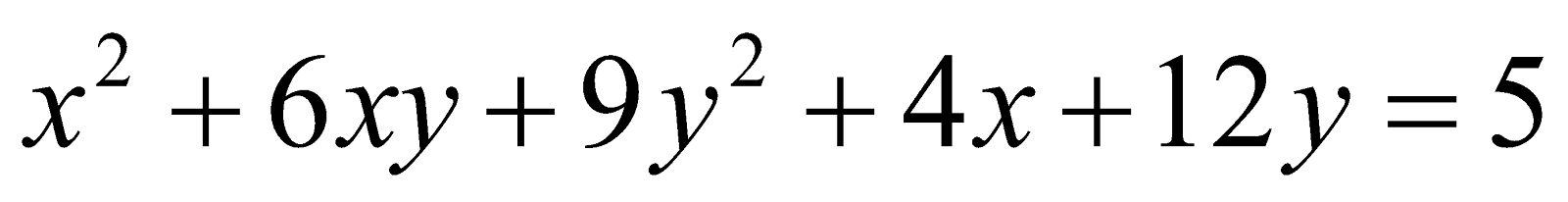represents a pair of straight lines.
(d) Show that the area of the triangle formed by the linesand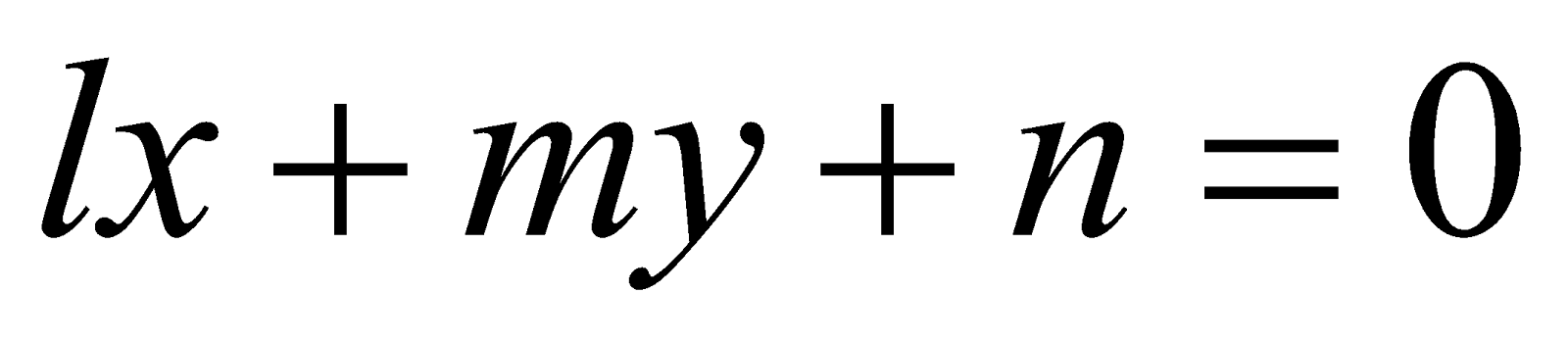is     5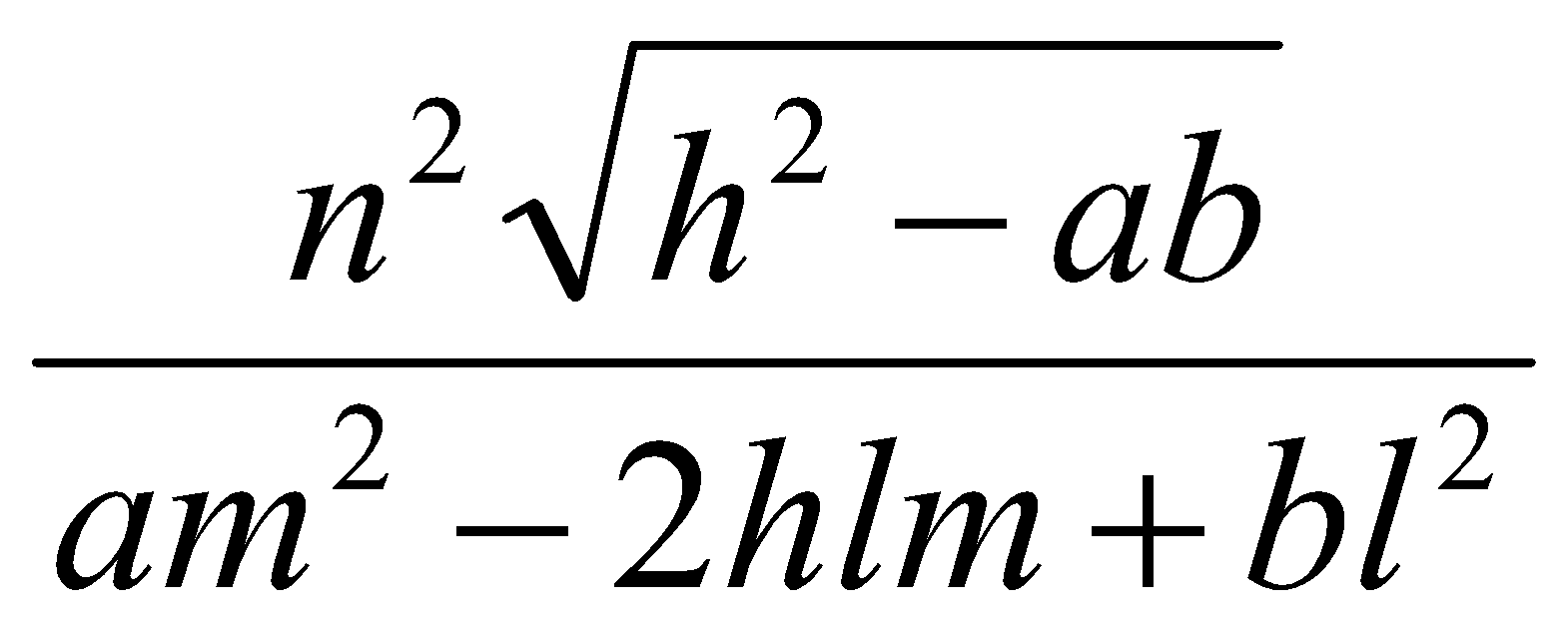Or
Show that the lines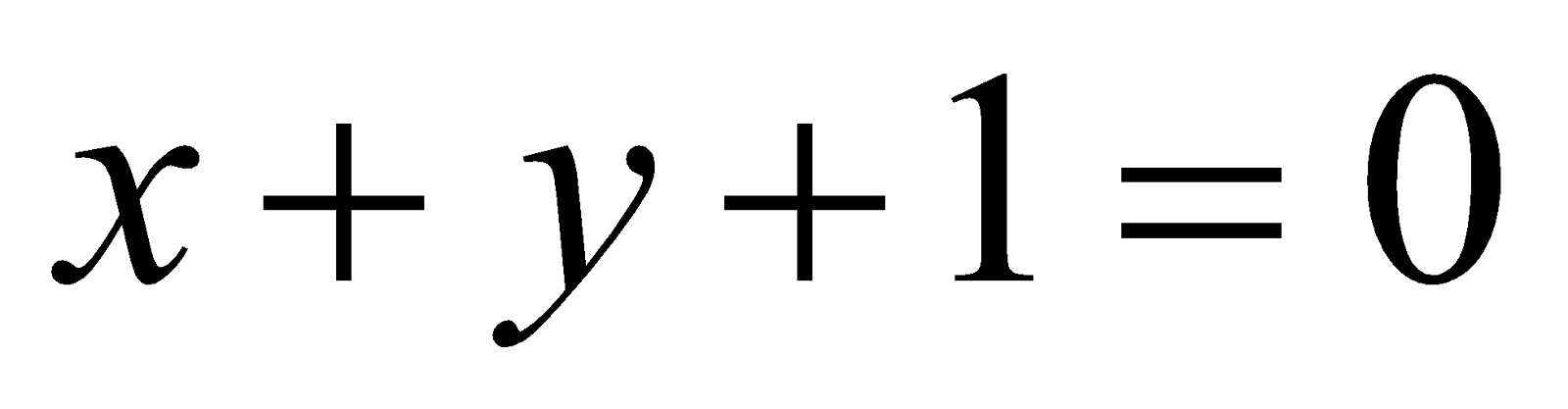and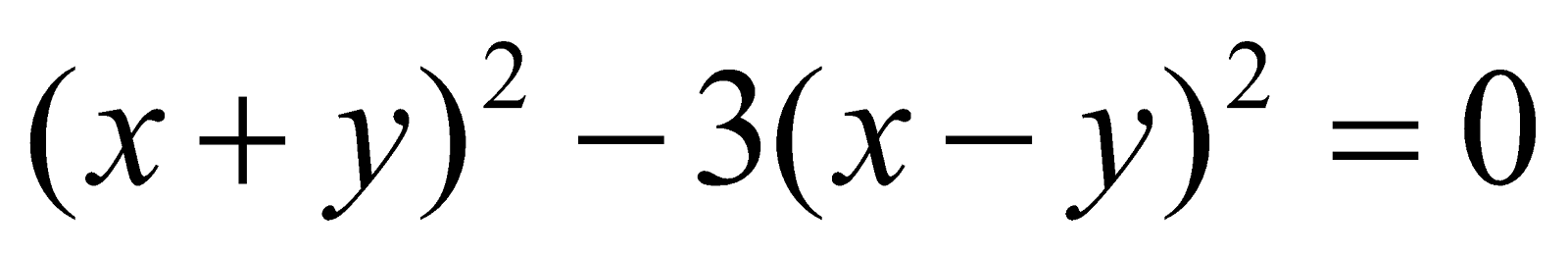form an equilateral triangle.
3. (a) Let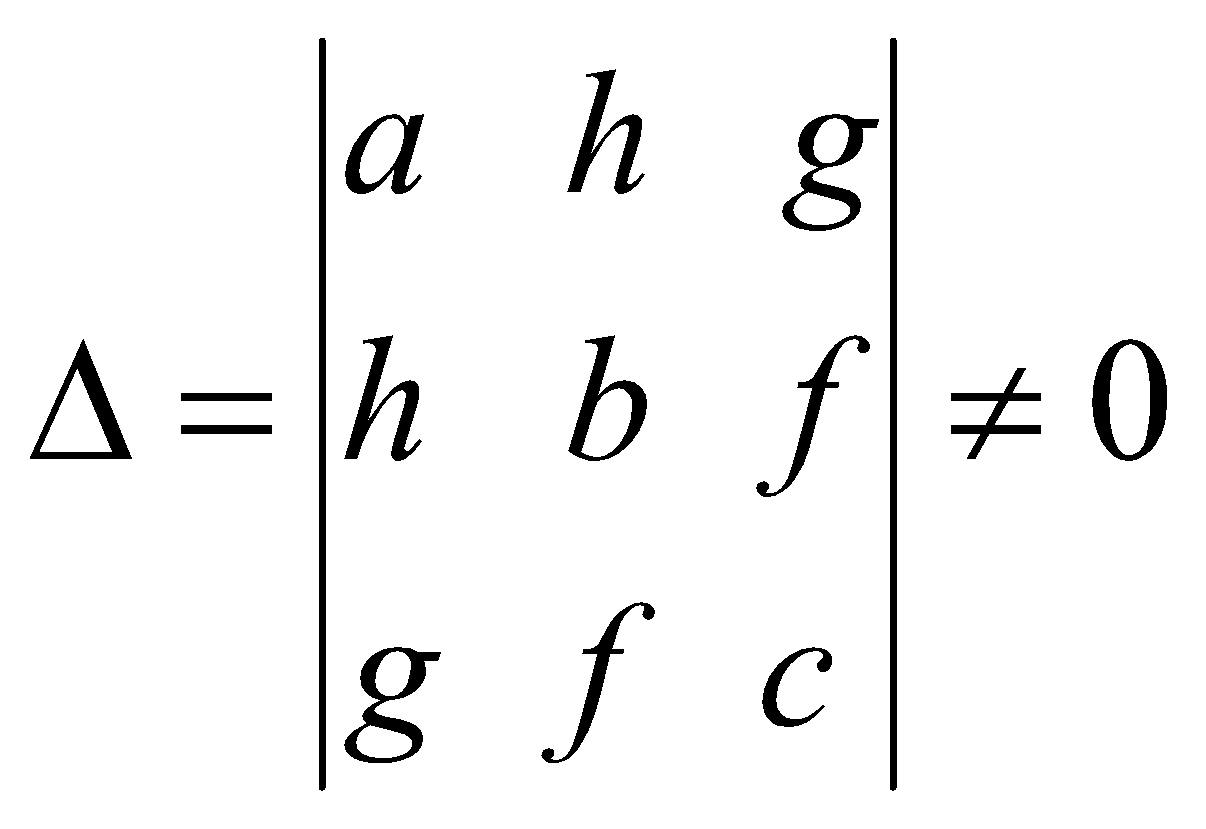and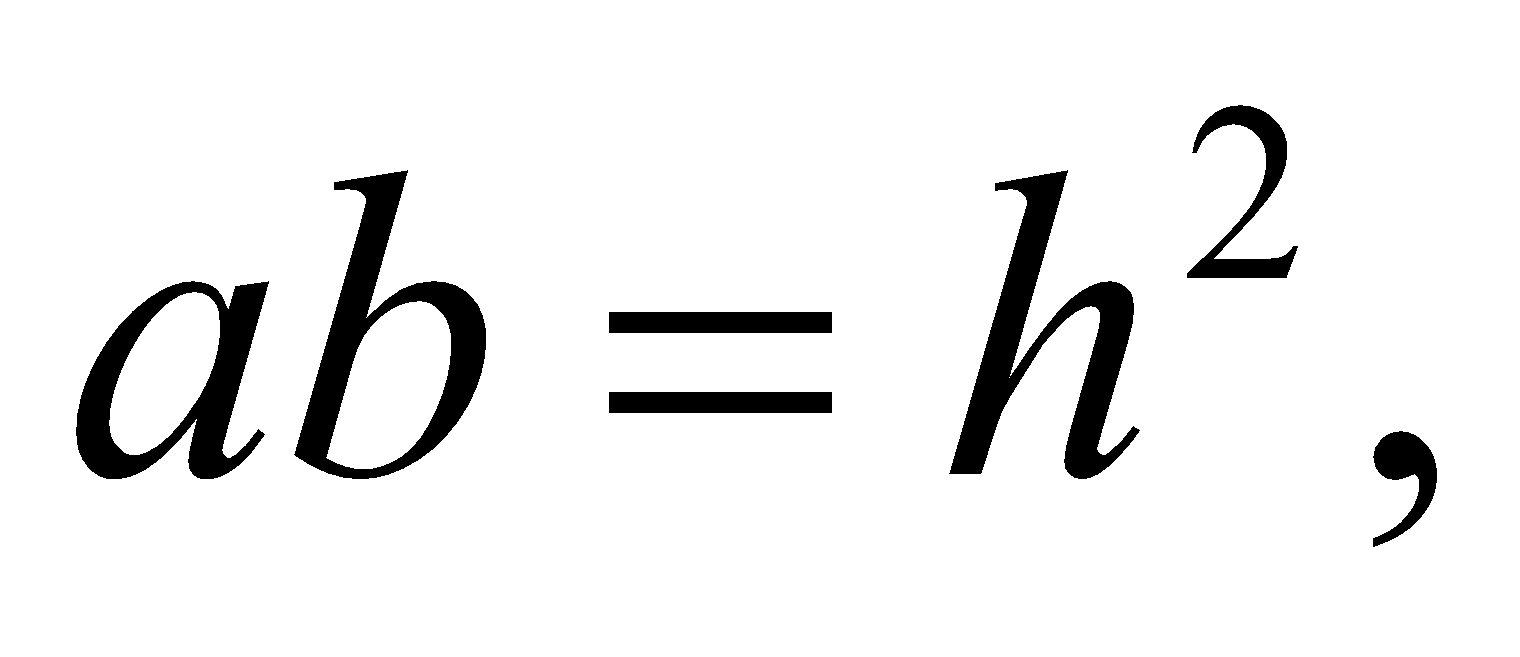for the conic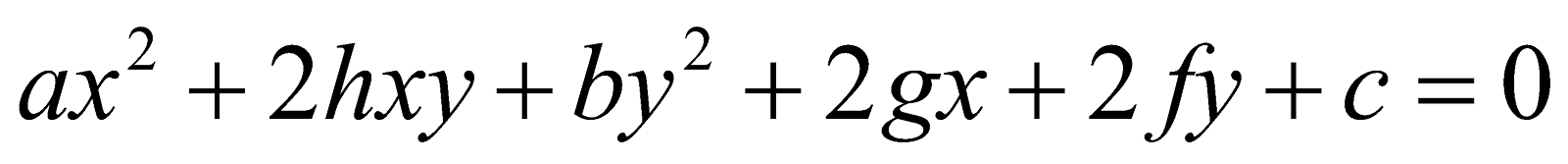. Write the name of the conic. 1
(b)  Find the equation of the tangent at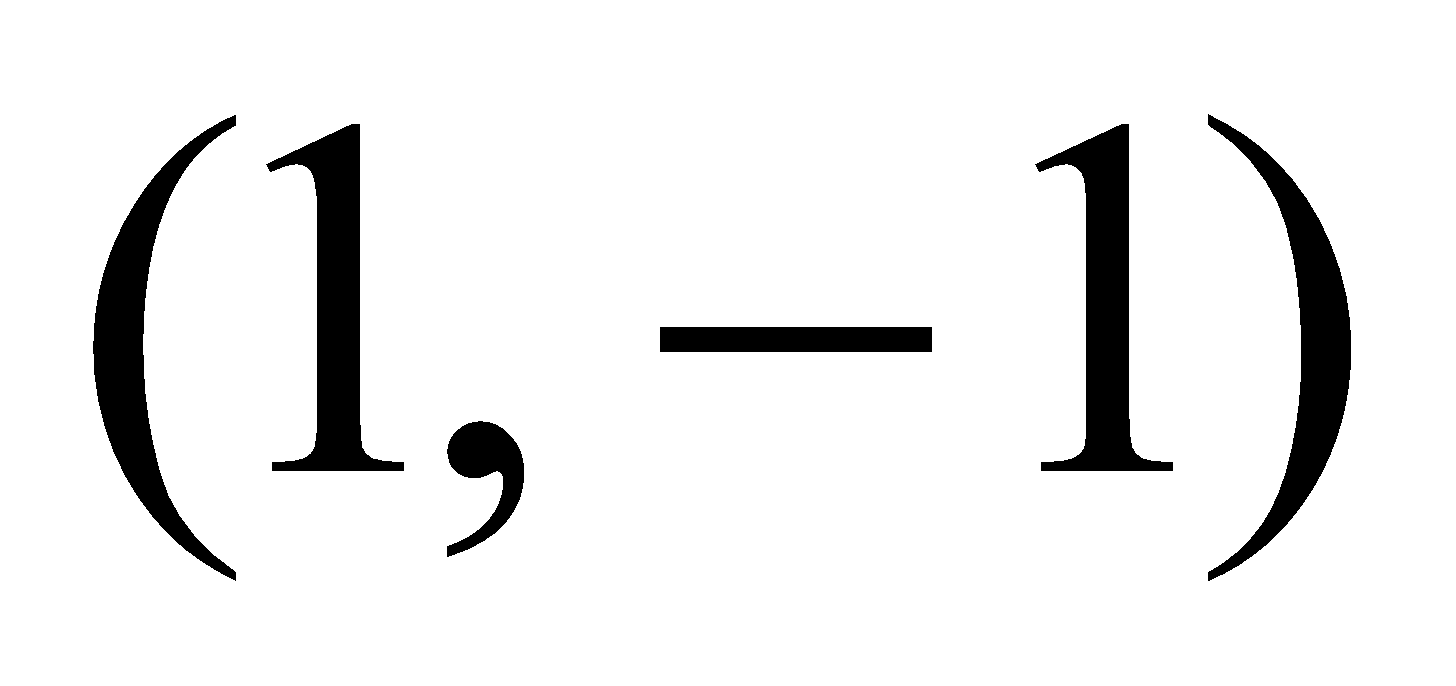to the conic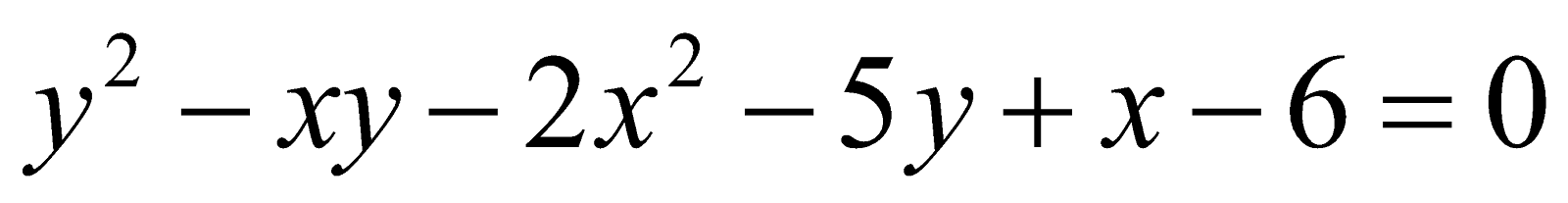. 2
(c) Write the definition of a diameter of a conic. 1
(d) Show that the equation 3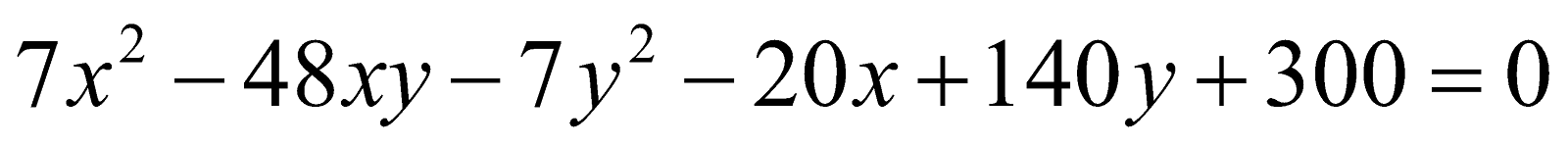represents a hyperbola.
(e) Show that the sum of the squares of two conjugate semi-diameters of a conic is constant. 3
Or
Determine the nature of the conic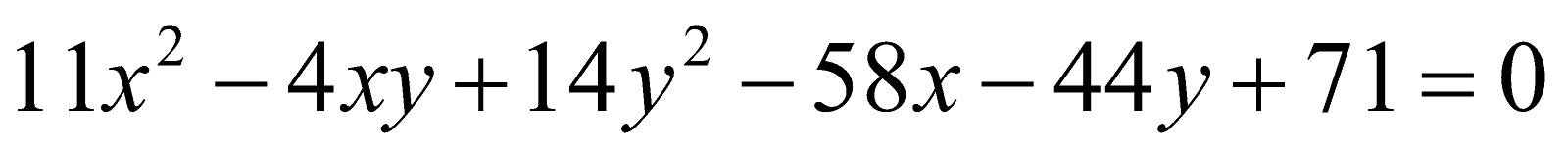. Also find the centre of the conic.
SECTION – II
(3-Dimension)
4. (a) Find the intercepts made by the plane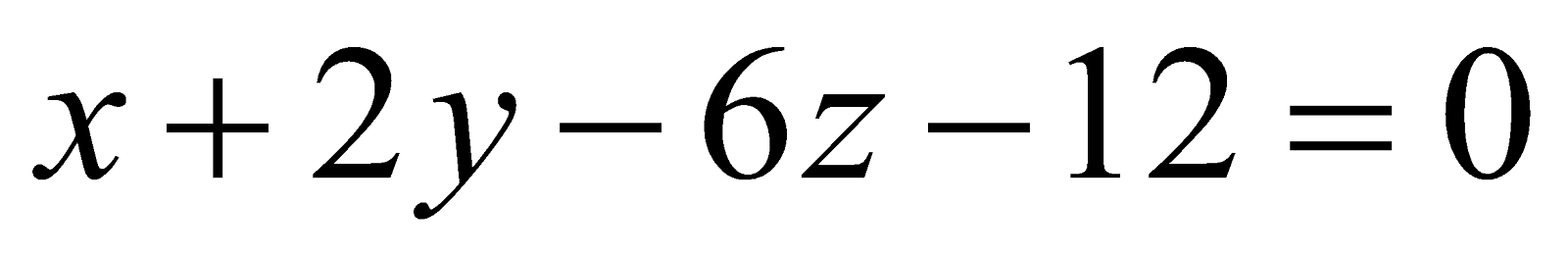on the axes. 1
(b) Express the equation of the plane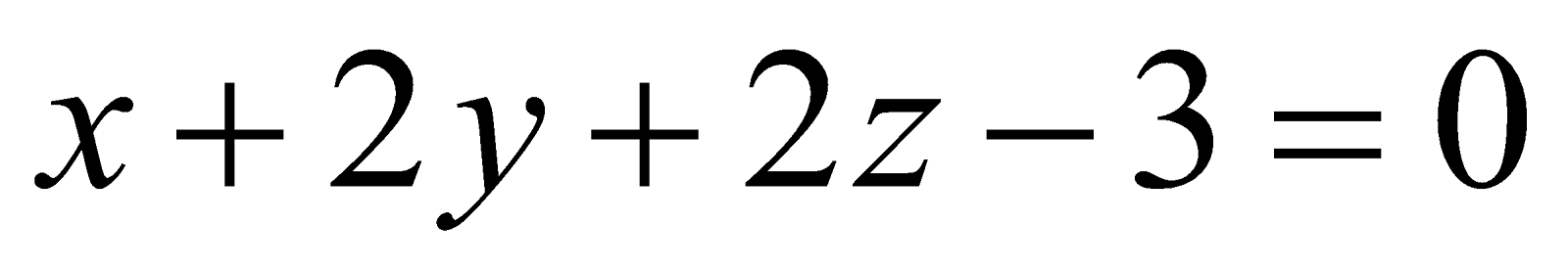in normal form. 2
(c) Find the equation to the plane through the point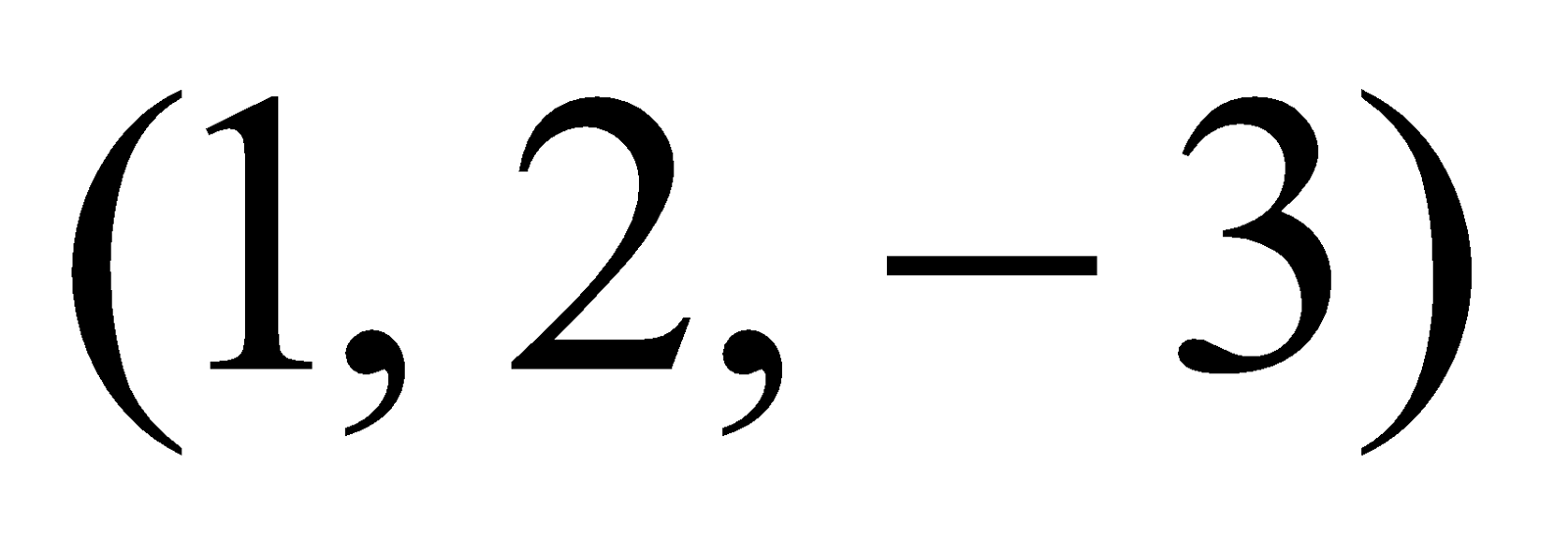and normal to the straight line joining the points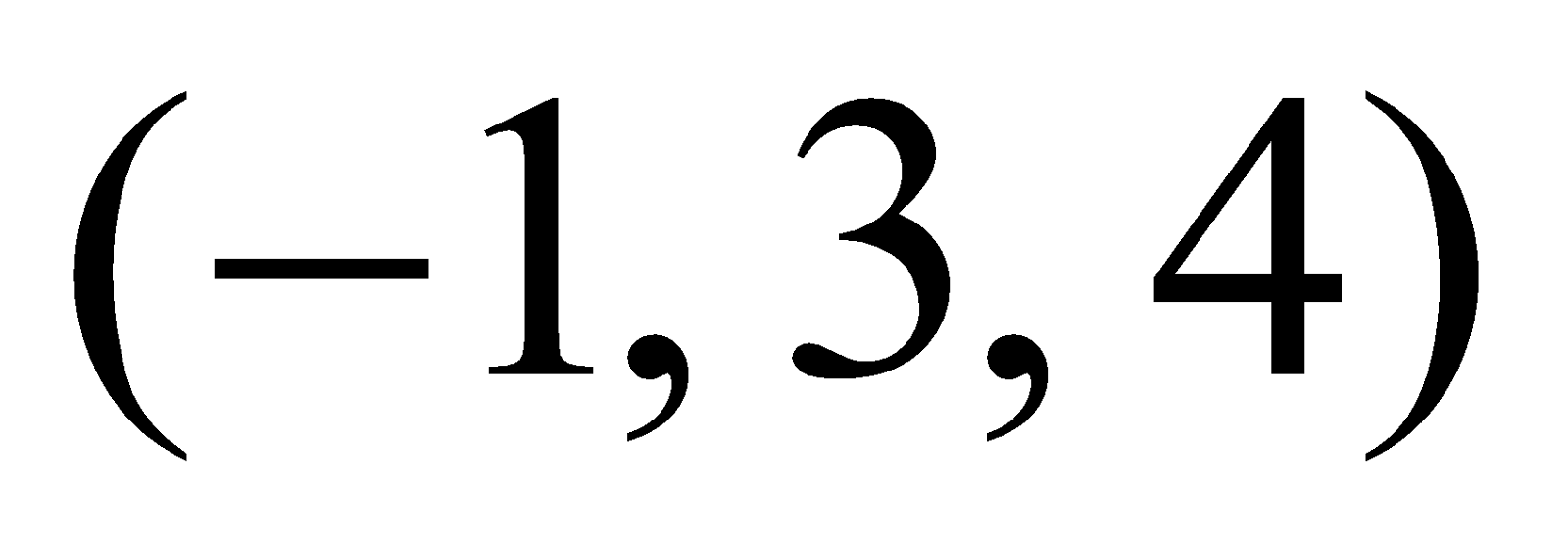and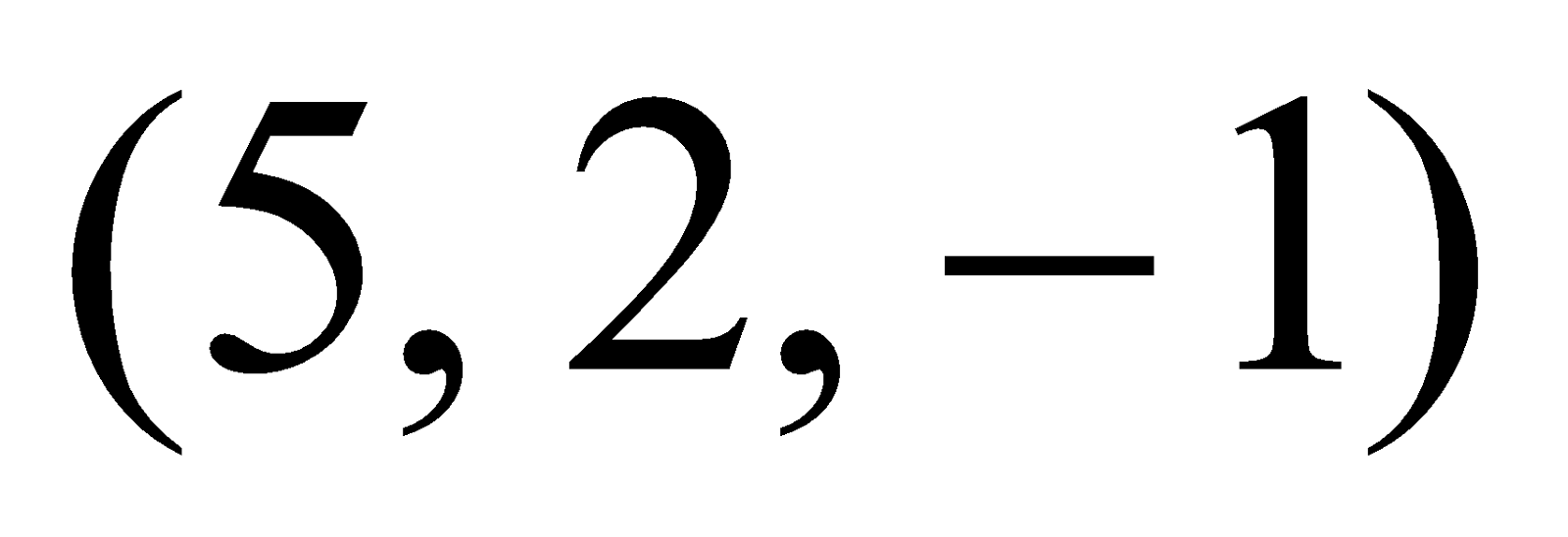. 3
Or
Find the coordinates of the point, where the line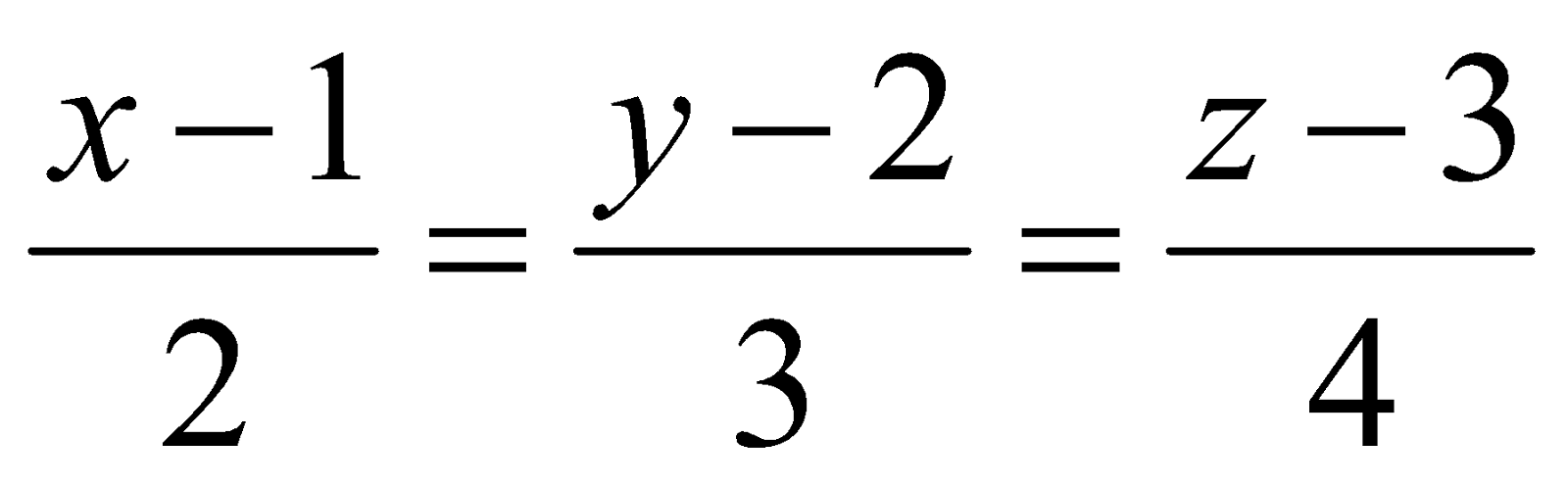meets the plane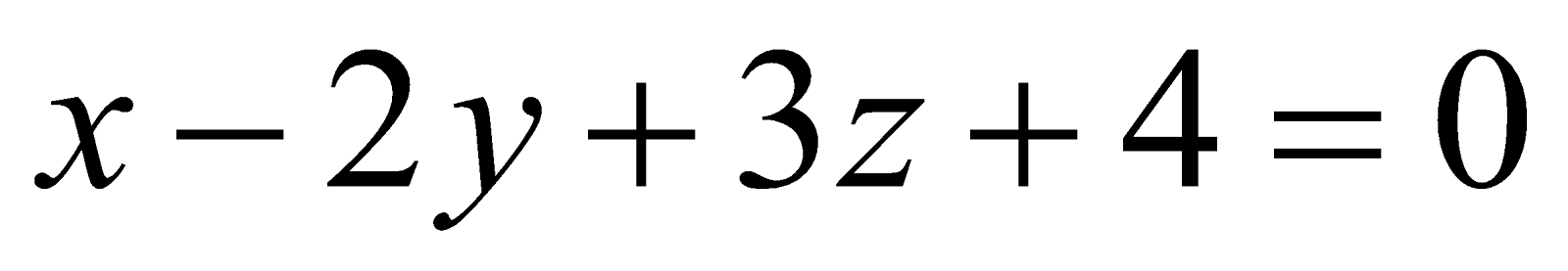.
(d) Find the distance of the point of intersection of the line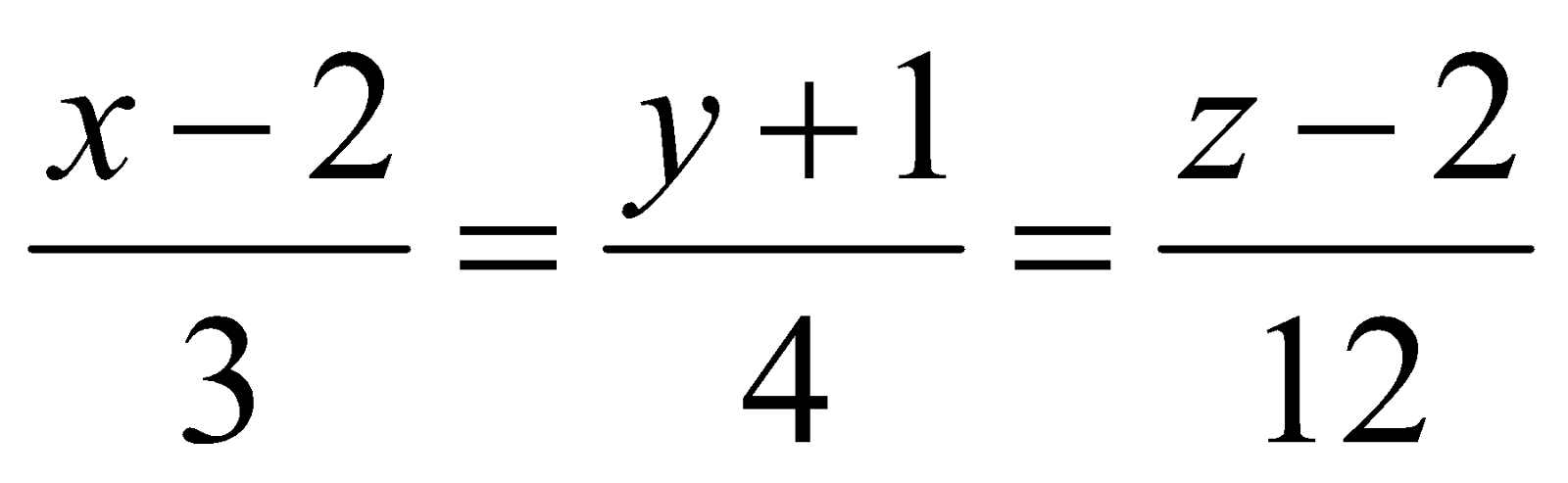and the plane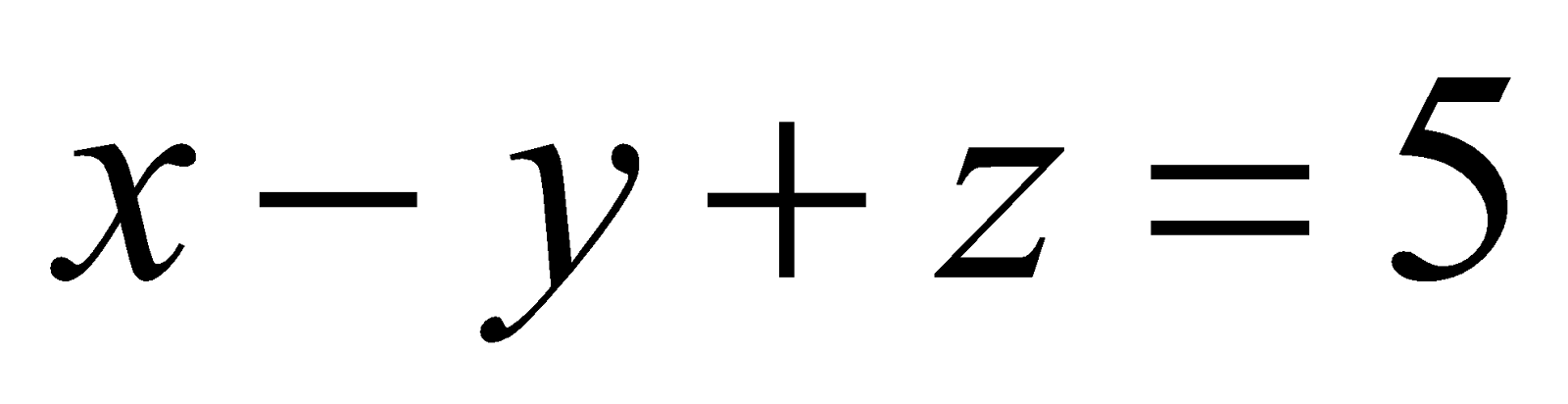from the point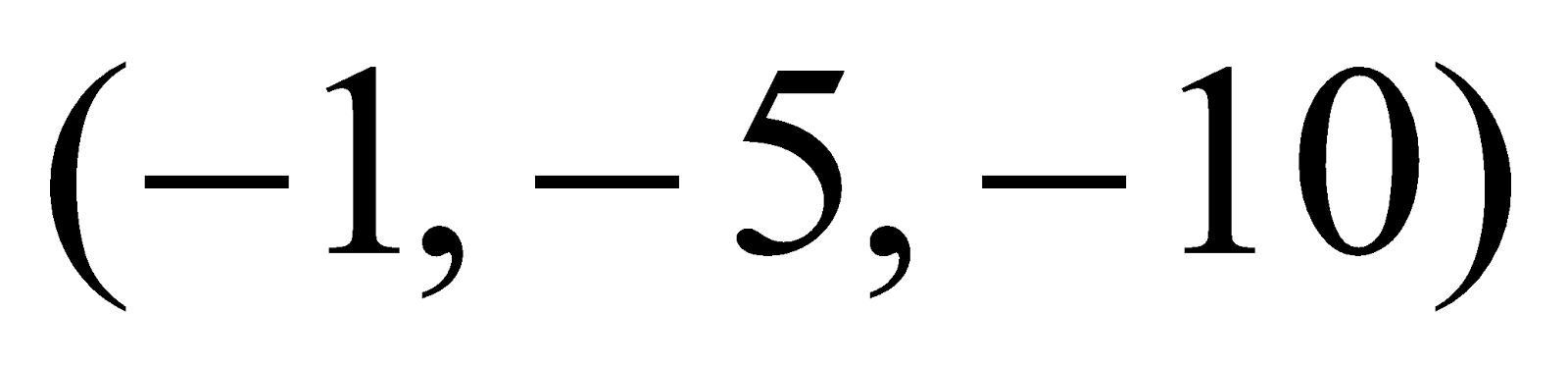.
5. (a) Define skew lines. 1
(b) Find the shortest distance between the planes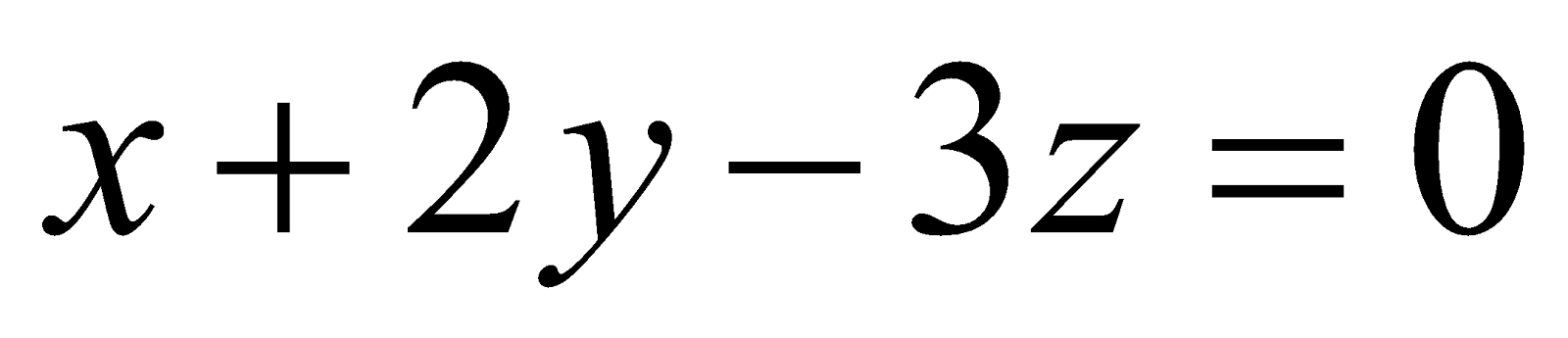and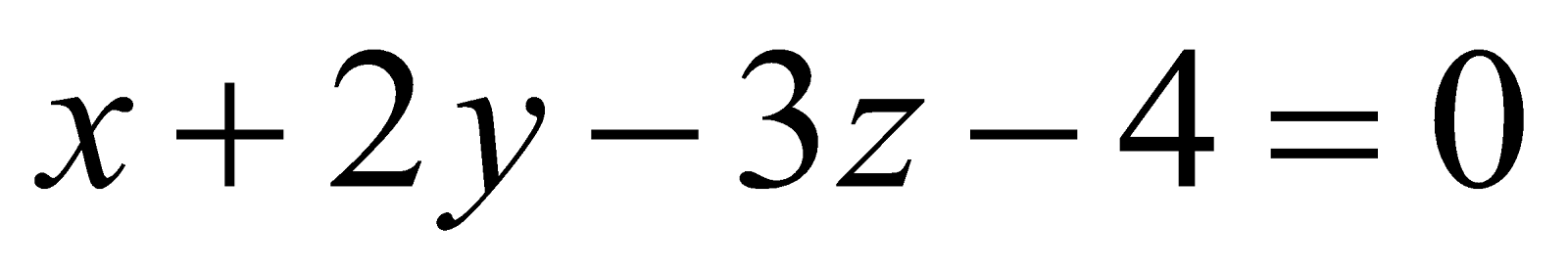. 2
(c) Find the shortest distance between the lines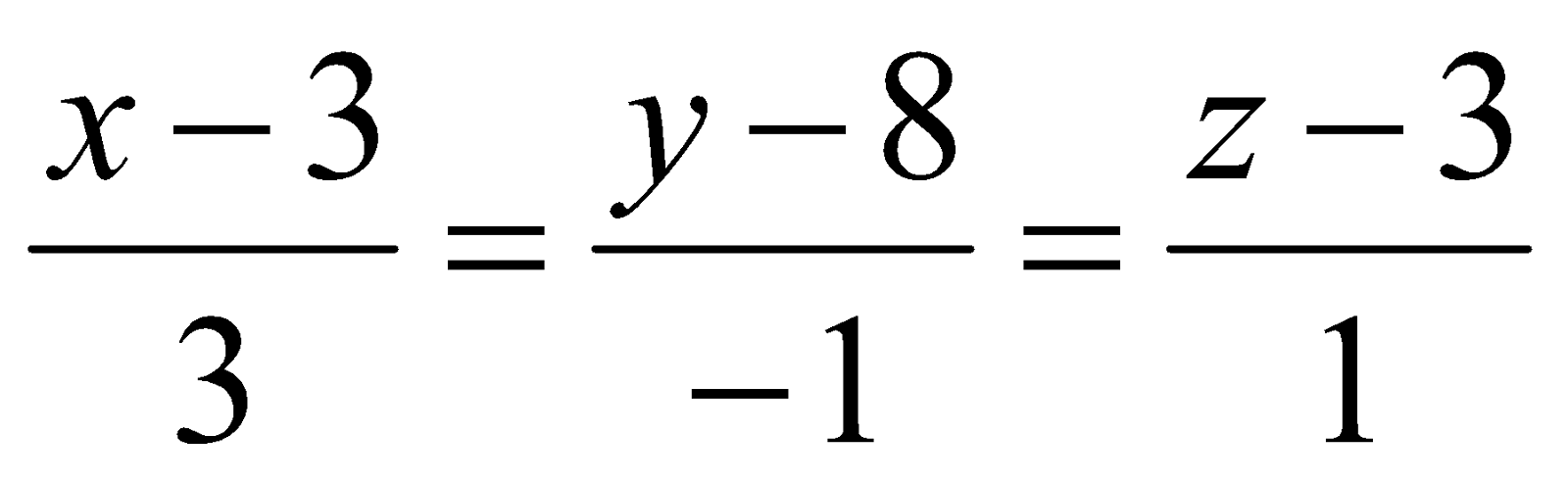and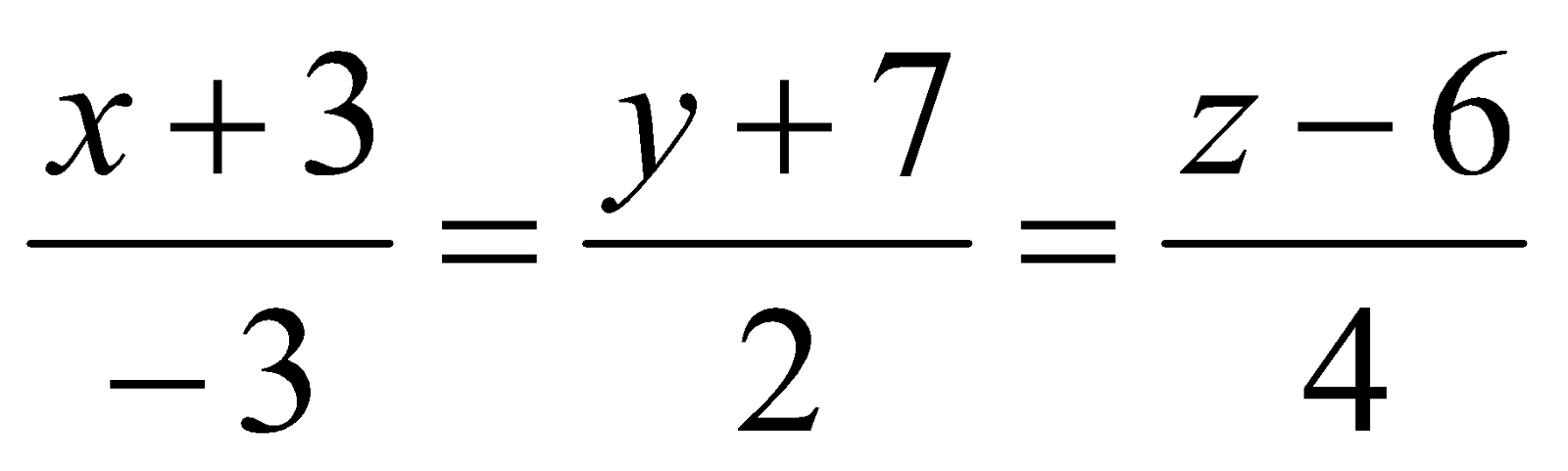. 5
GROUP – B
(Analysis – I)
6. (a) If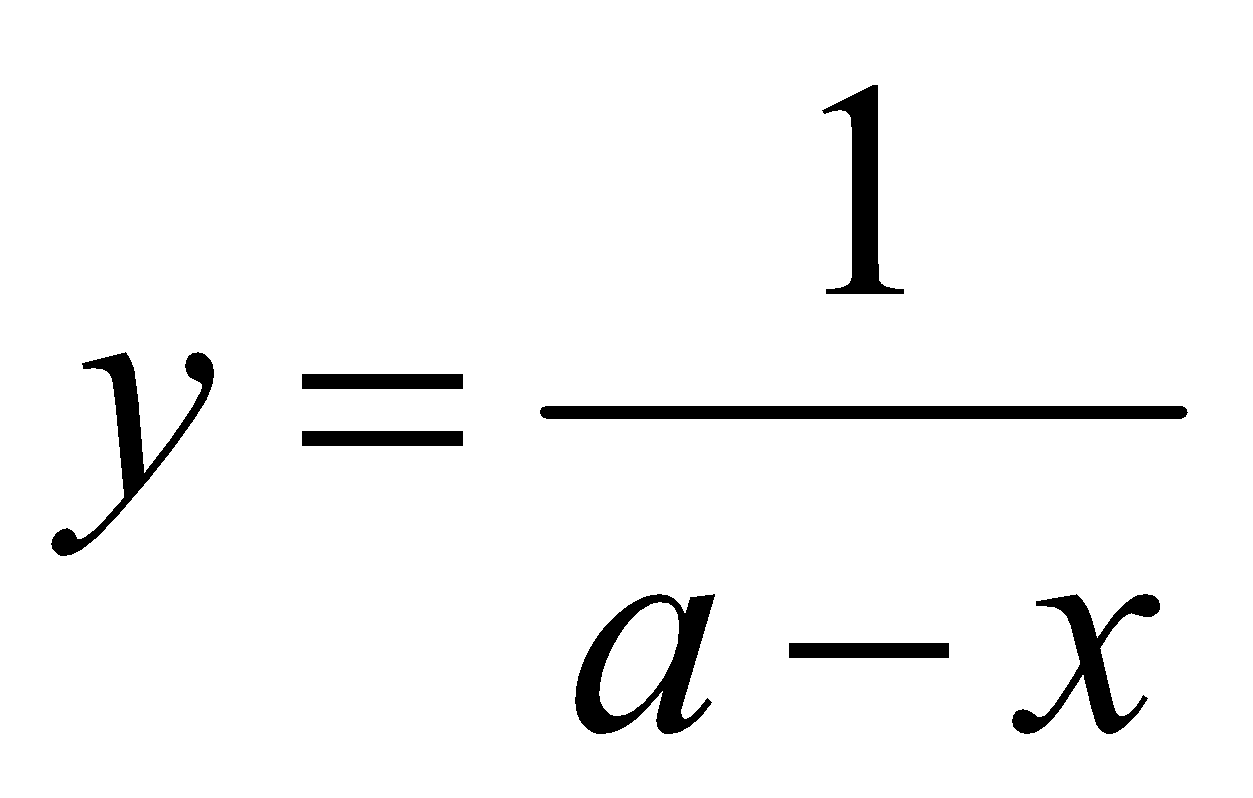, then write the value of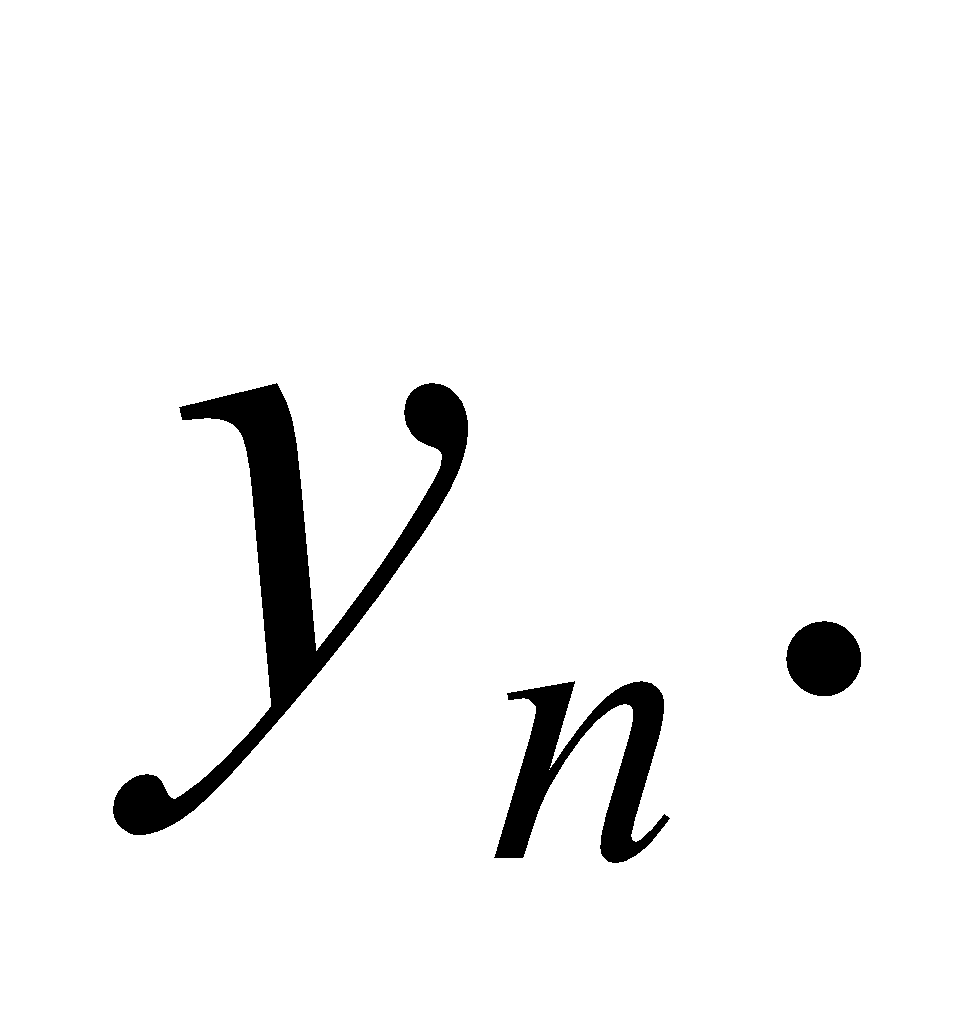1
(b) If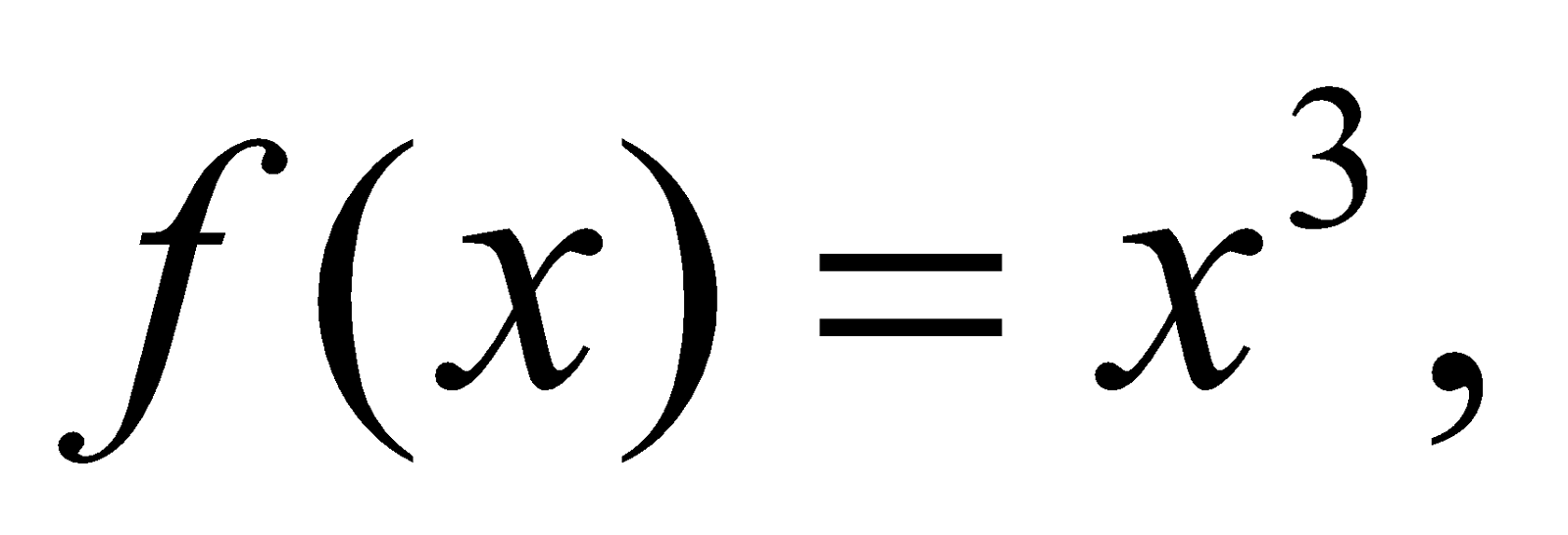then find the length of sub tangent of the curve. 1
(c) Find the radius of curvature at any point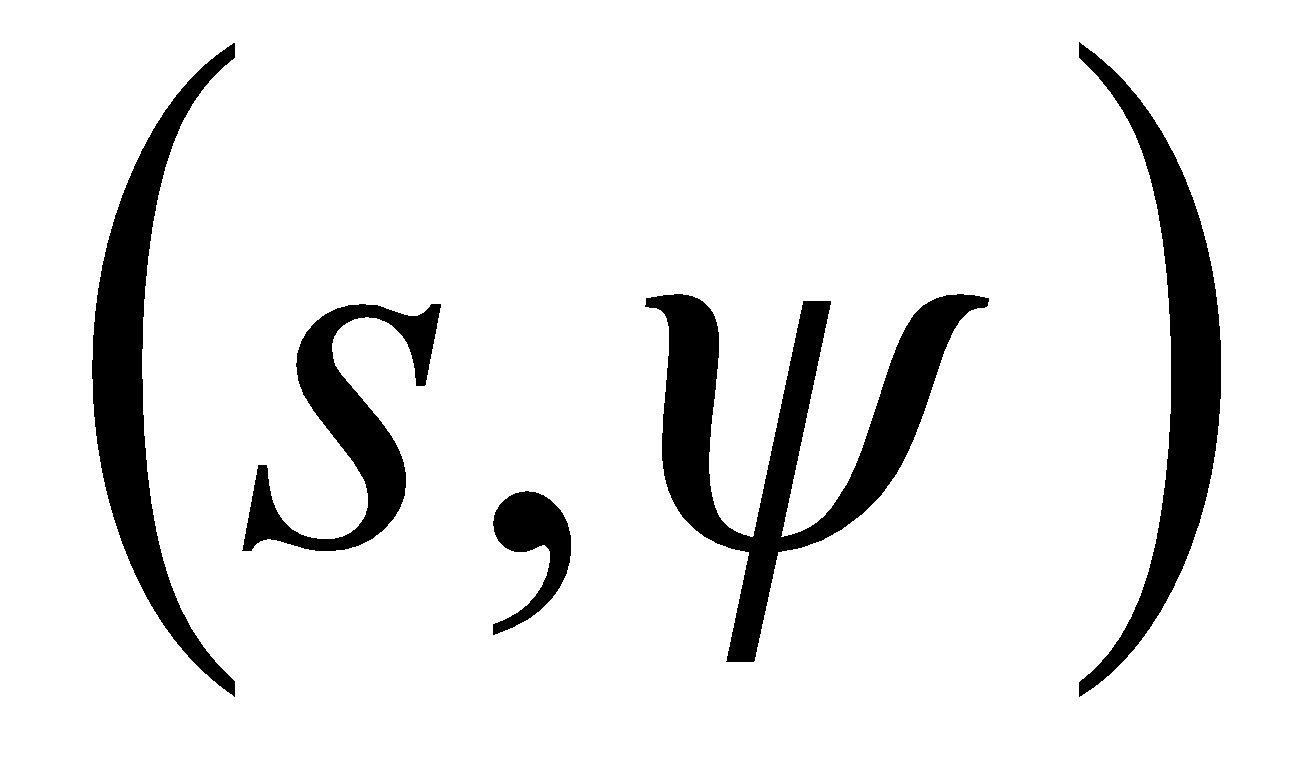on the curve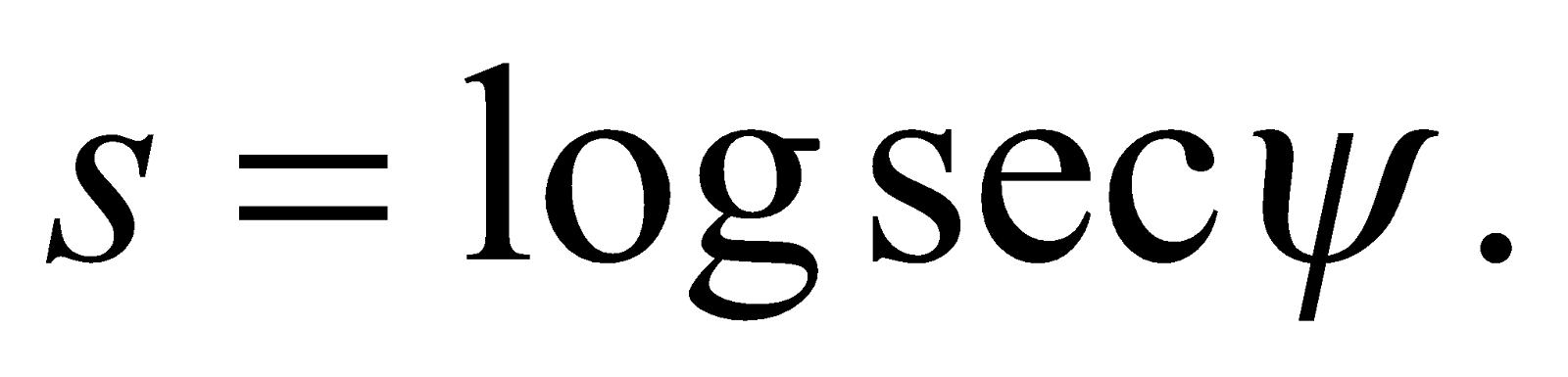2
(d) Ifthen find the value of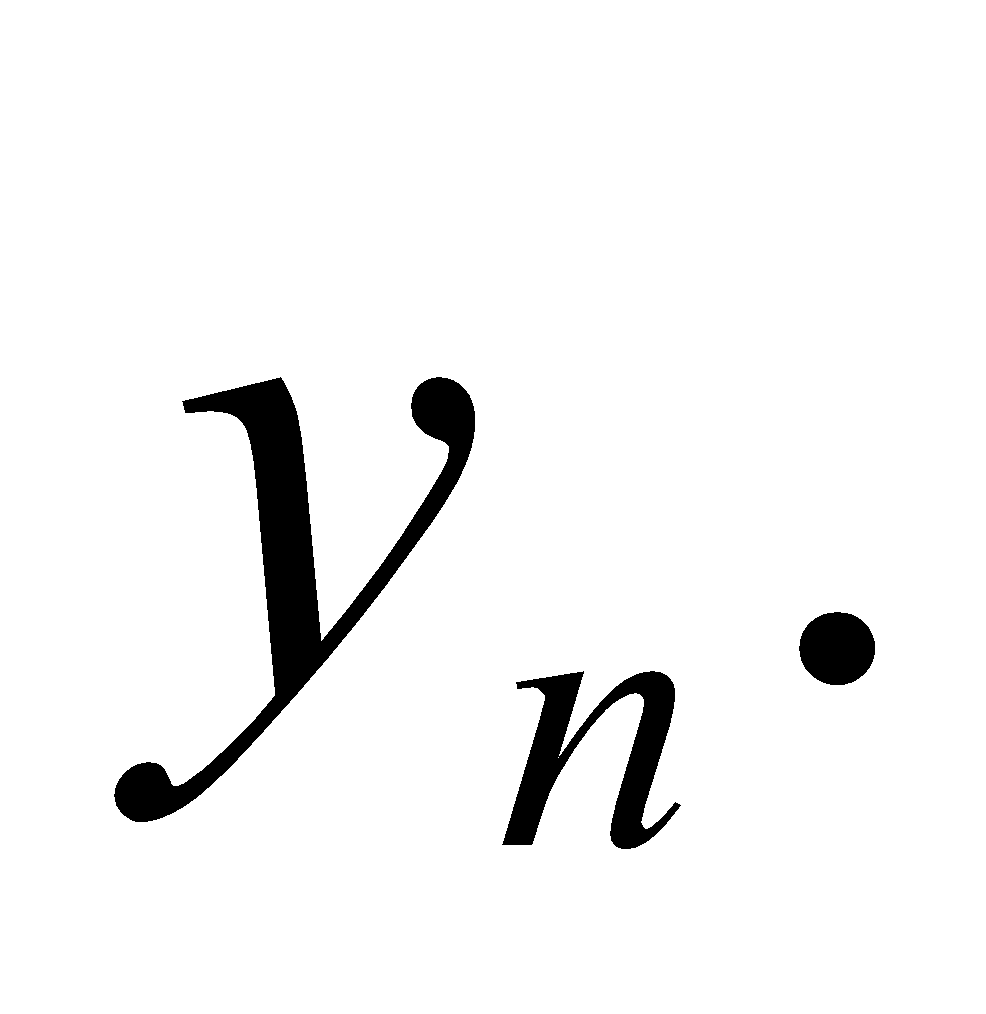3
(e) Evaluate: 3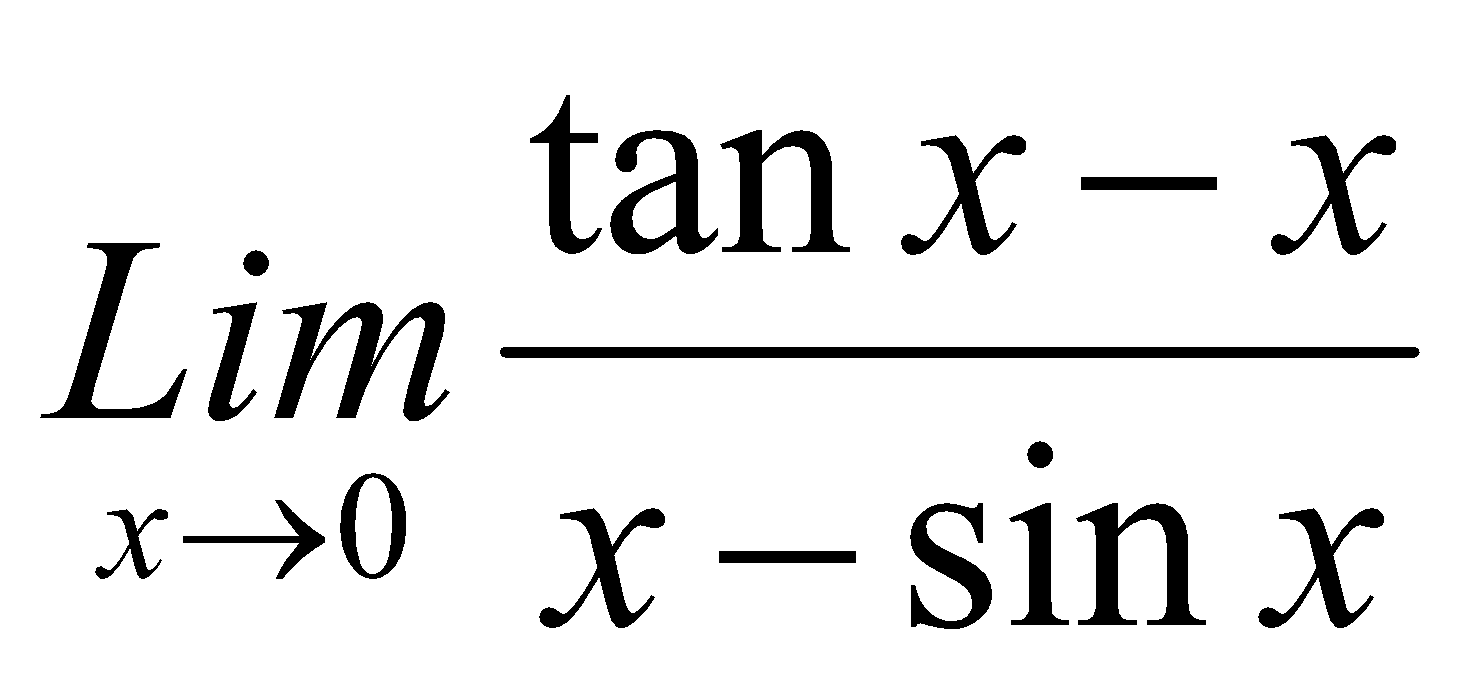Or
If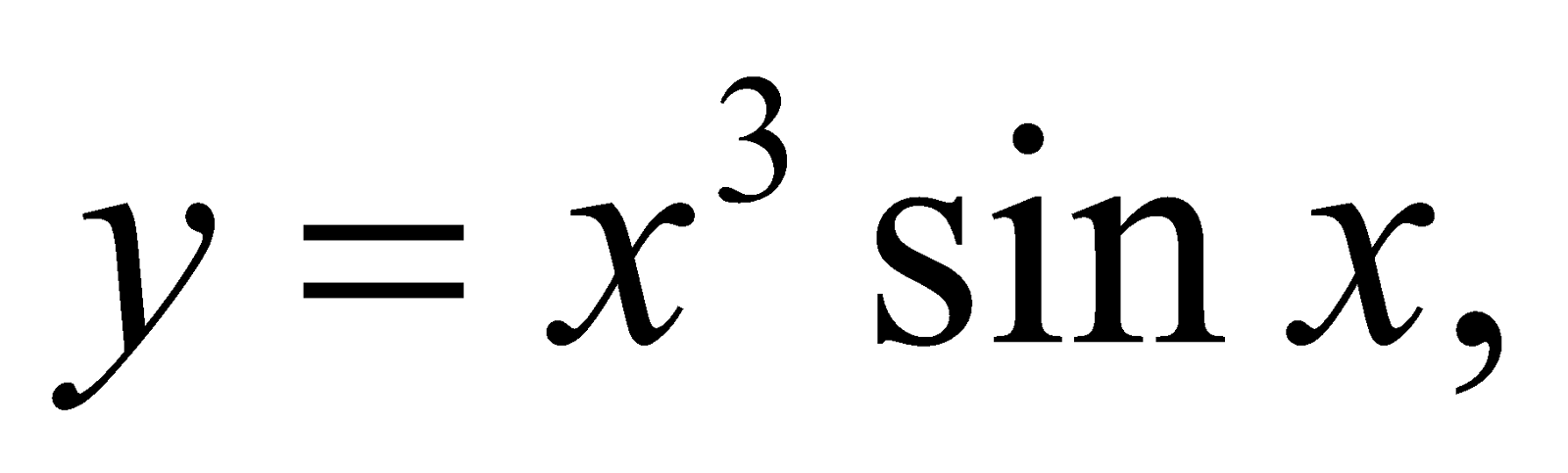then find the value of7. (a) Write when a function is derivable in a closed interval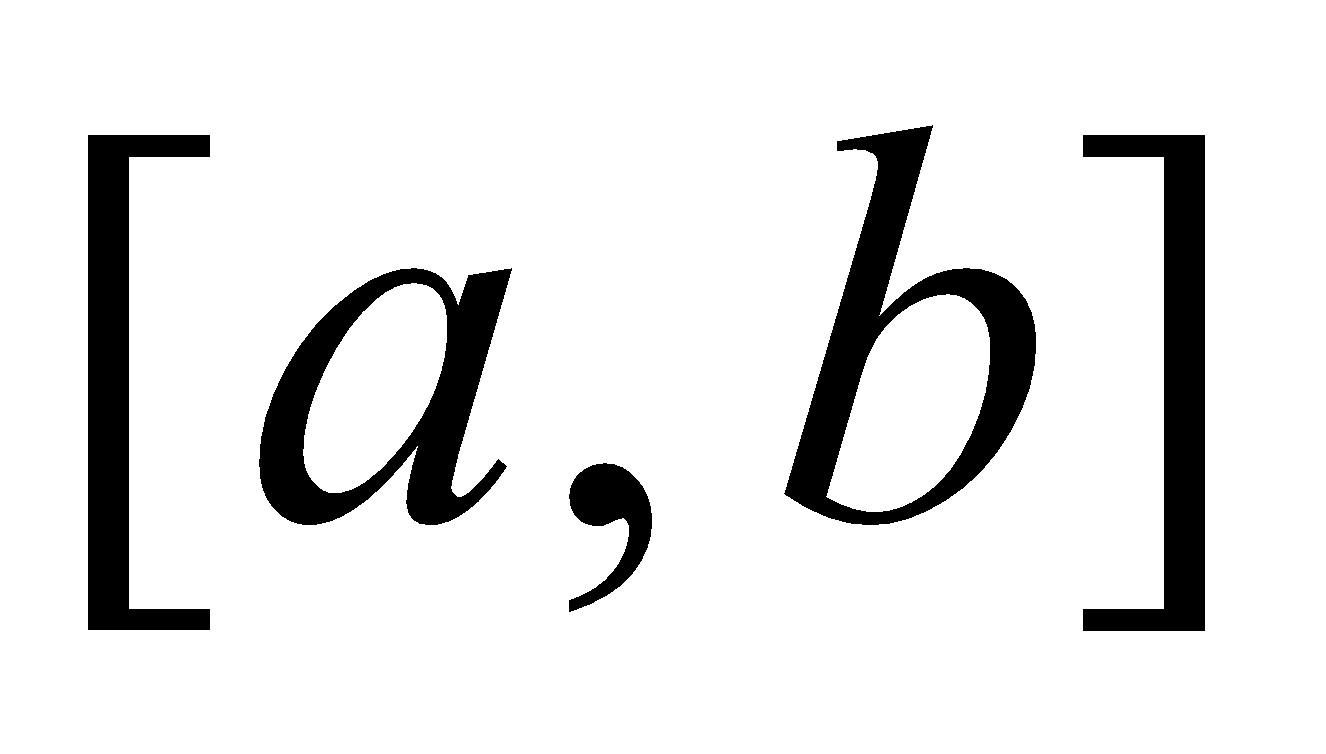. 1
(b) Write the geometrical interpretation of Lagrange mean value theorem. 2
(c) Prove that if a function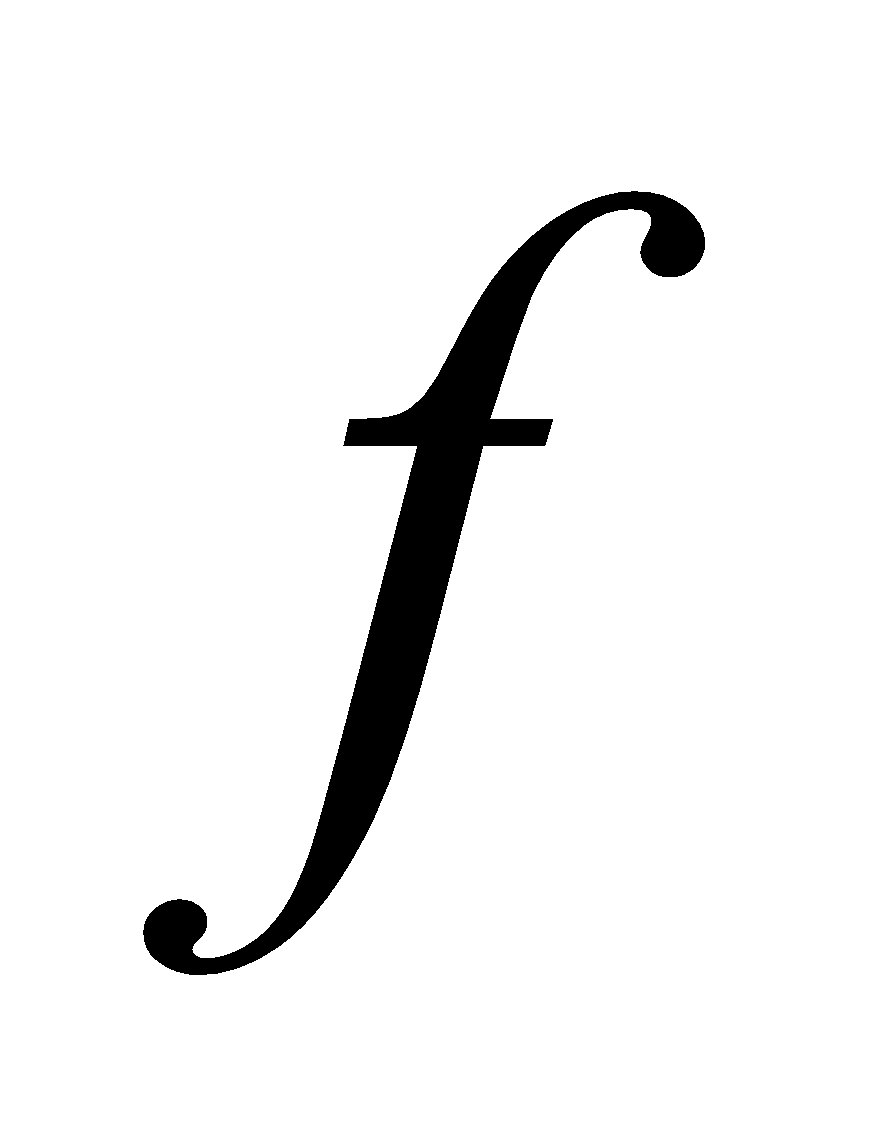is continuous onand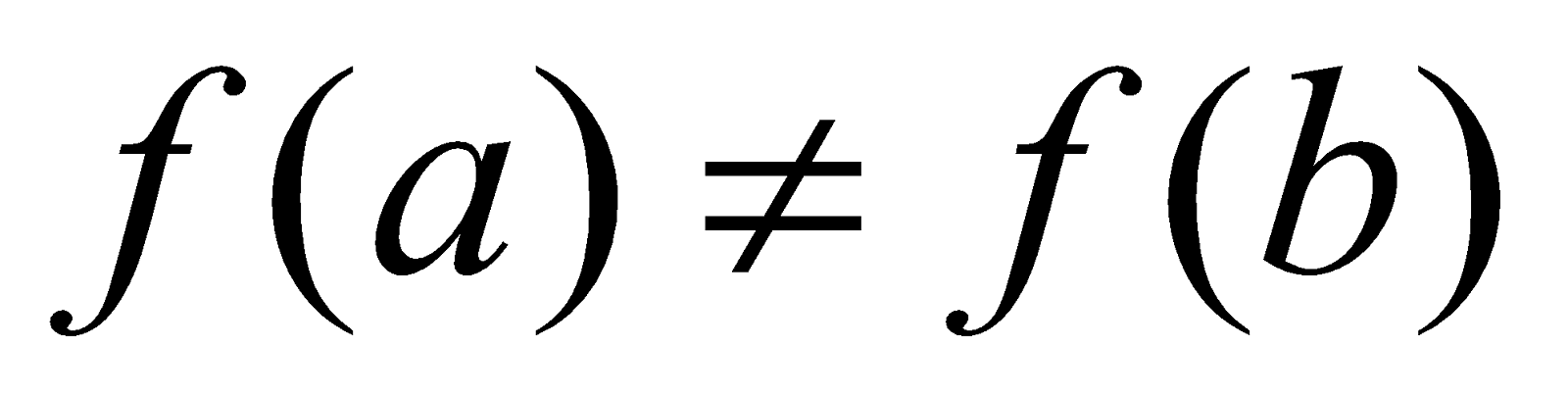, then it assumes every value between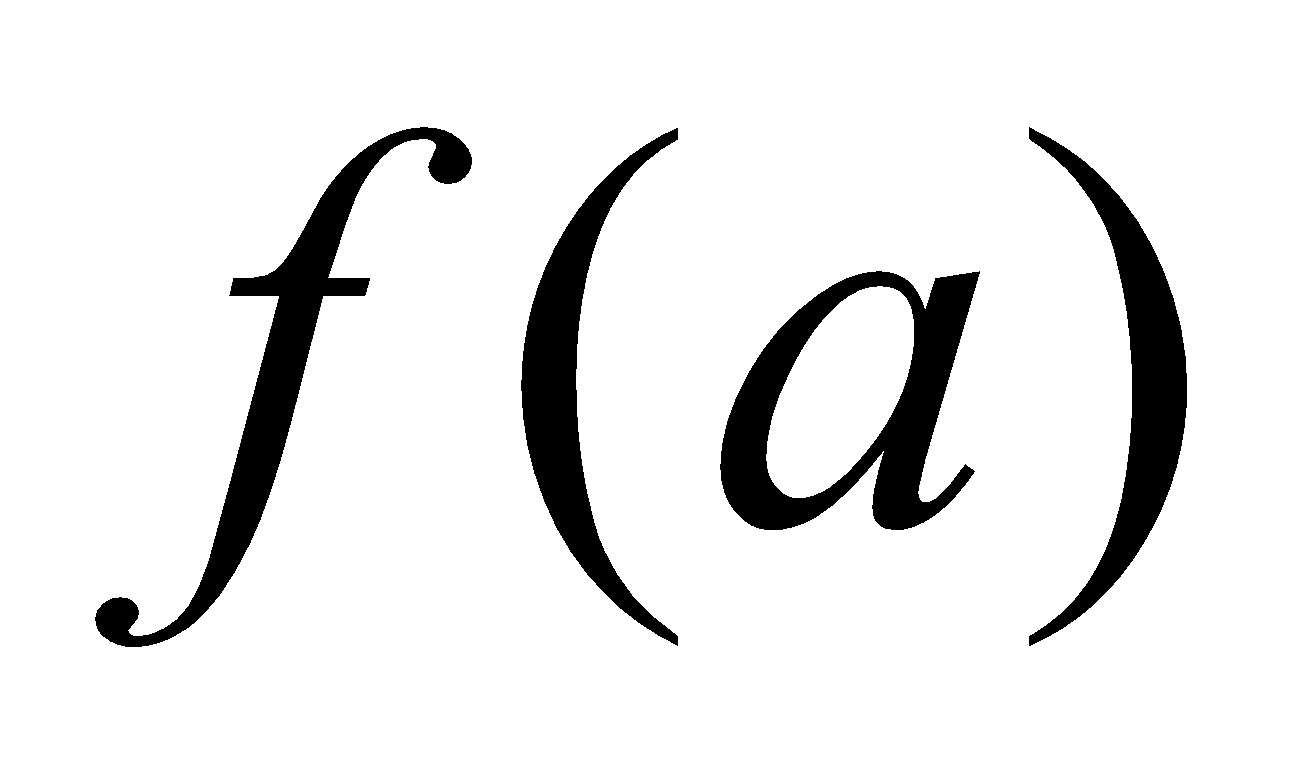and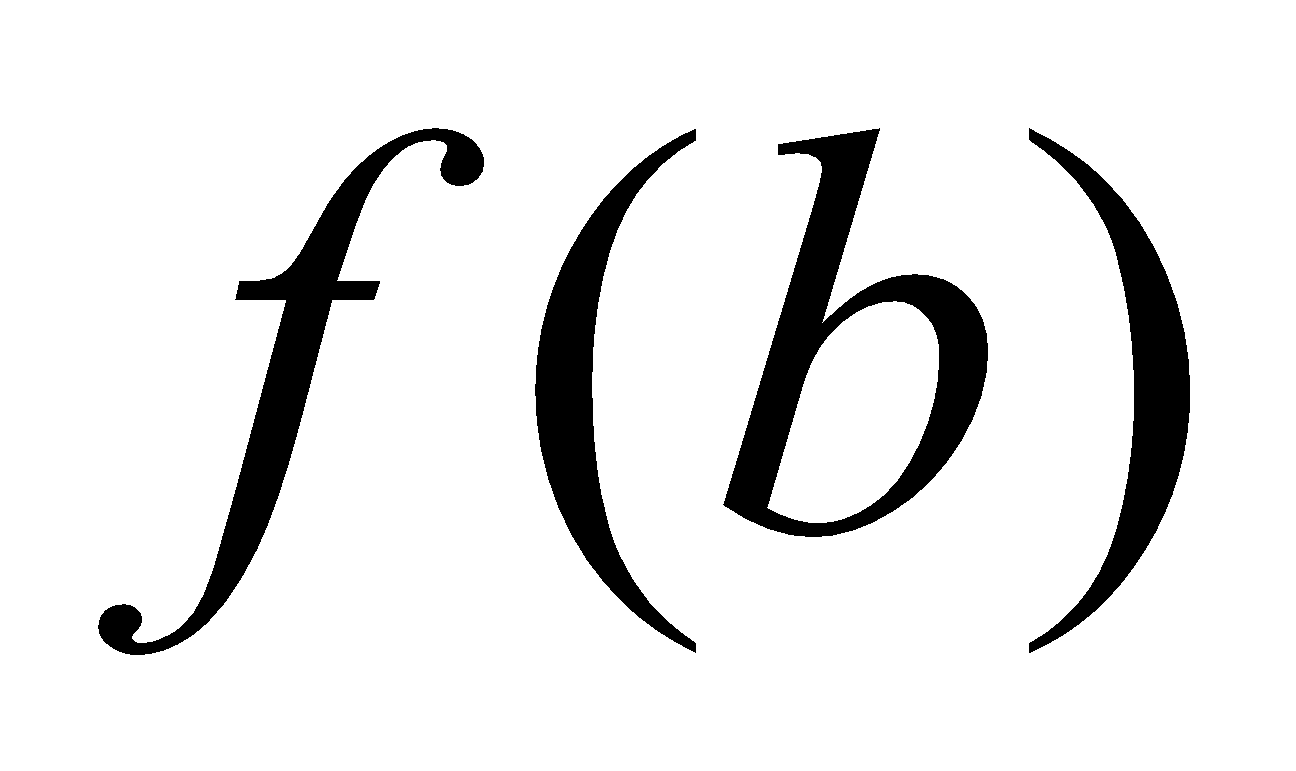. 4
(d) Discuss the applicability of Rolle’s Theorem to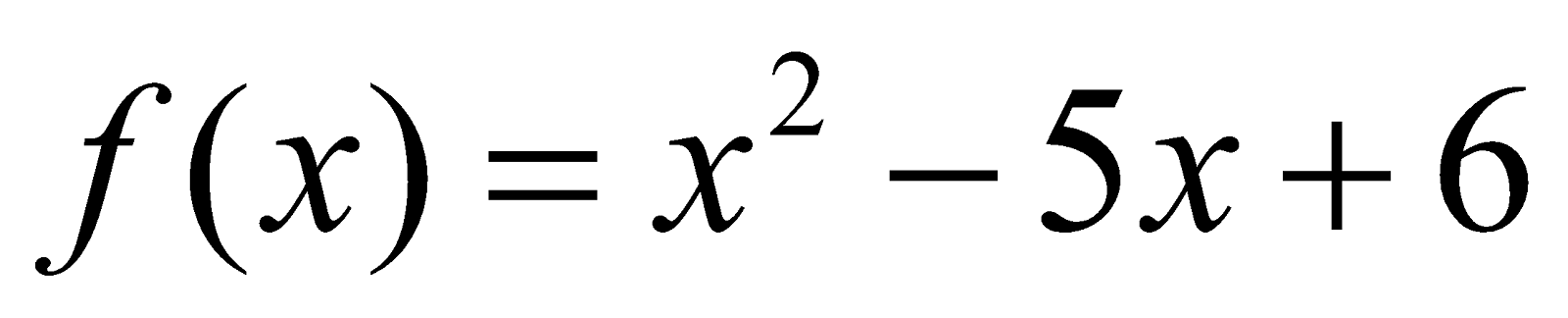in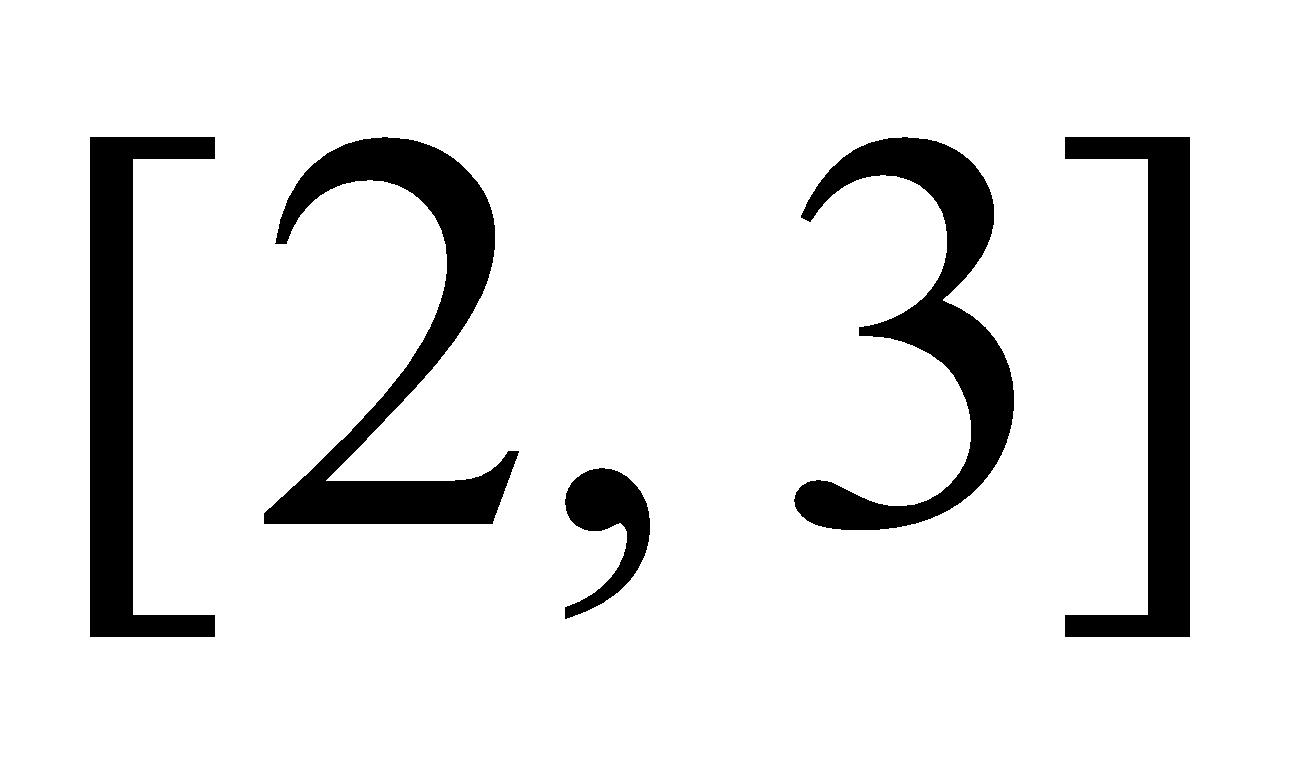. 3
Or
Expand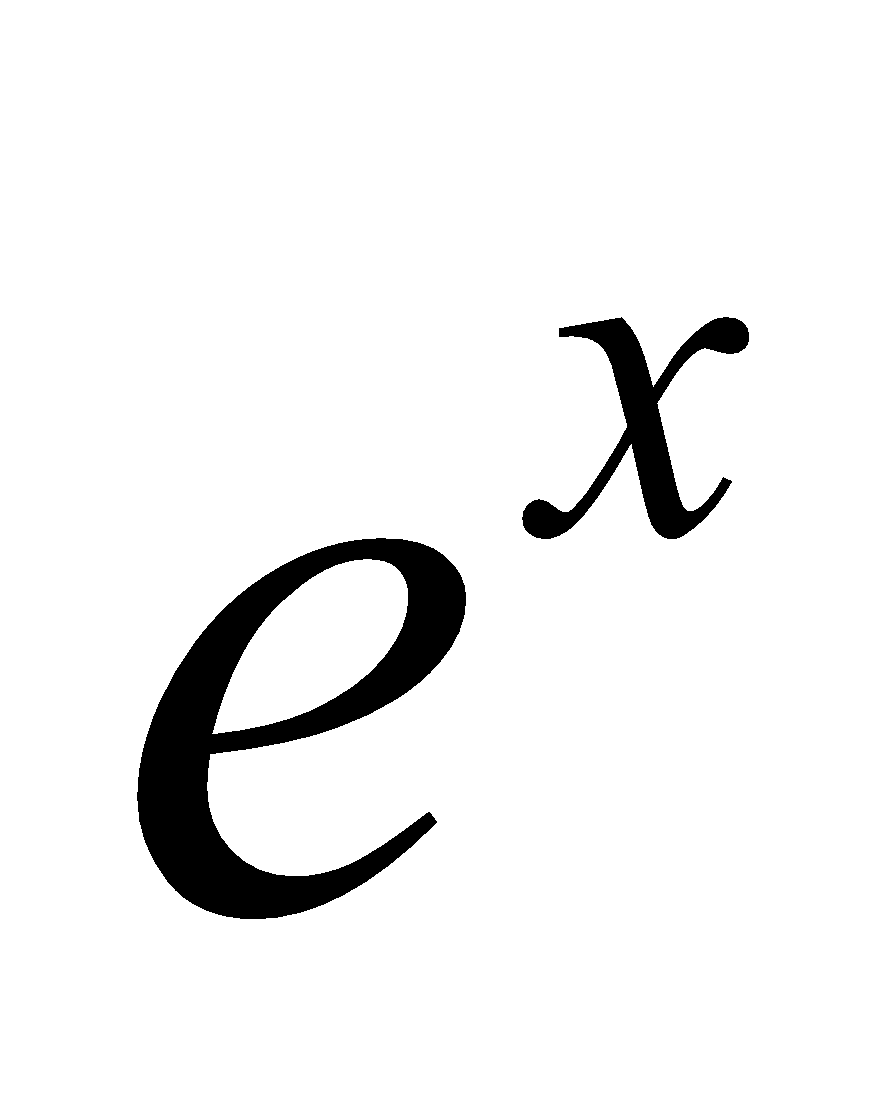by Maclaurin’s theorem with Lagrange form of remainder.
8. (a) If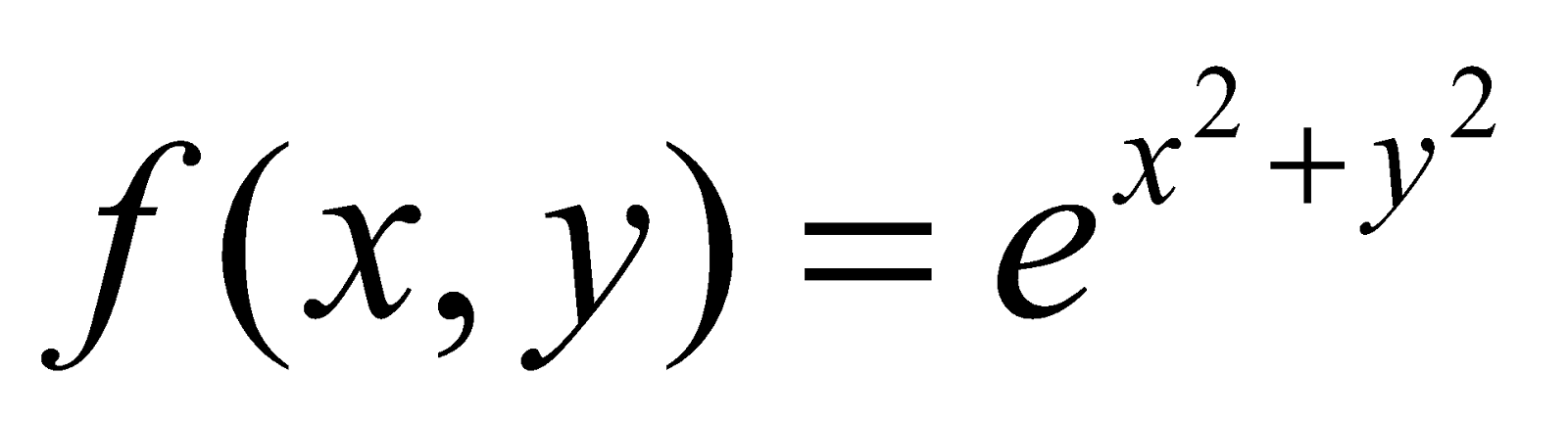, then find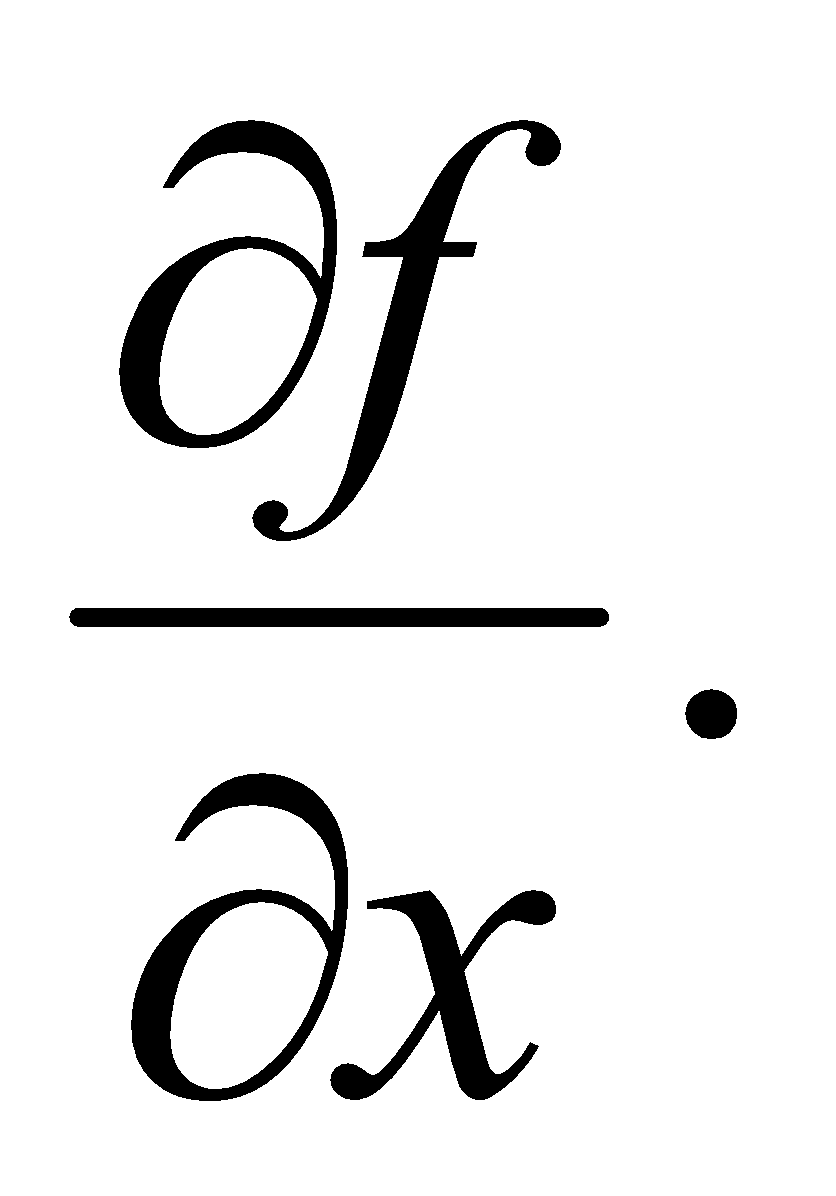2
(b) If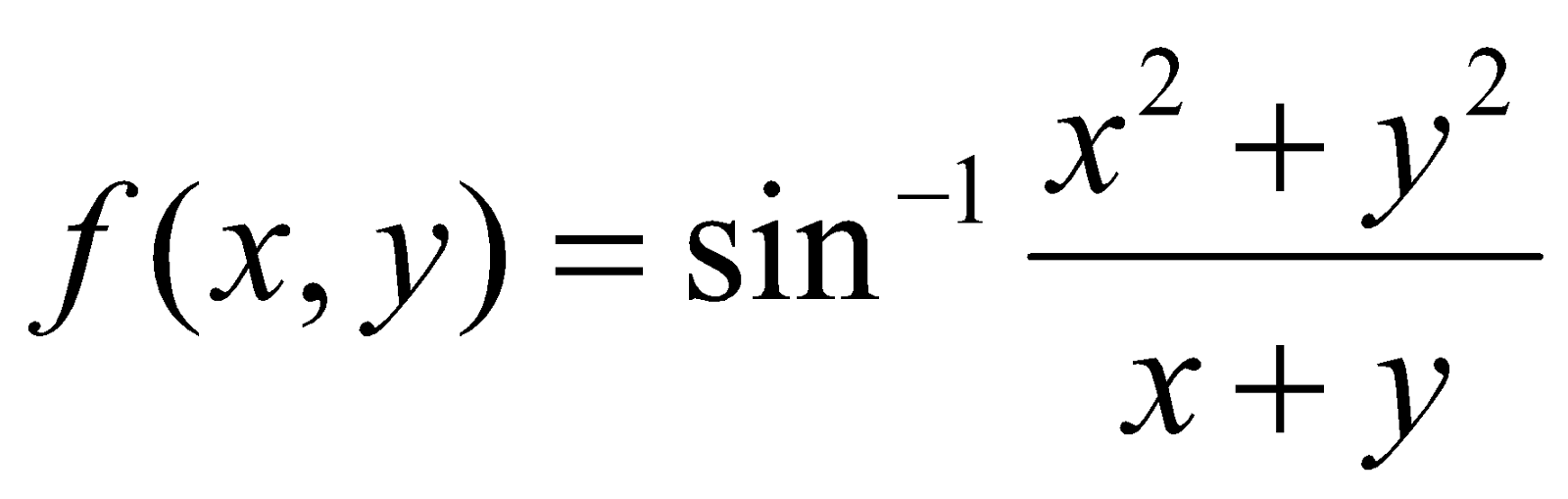, then show that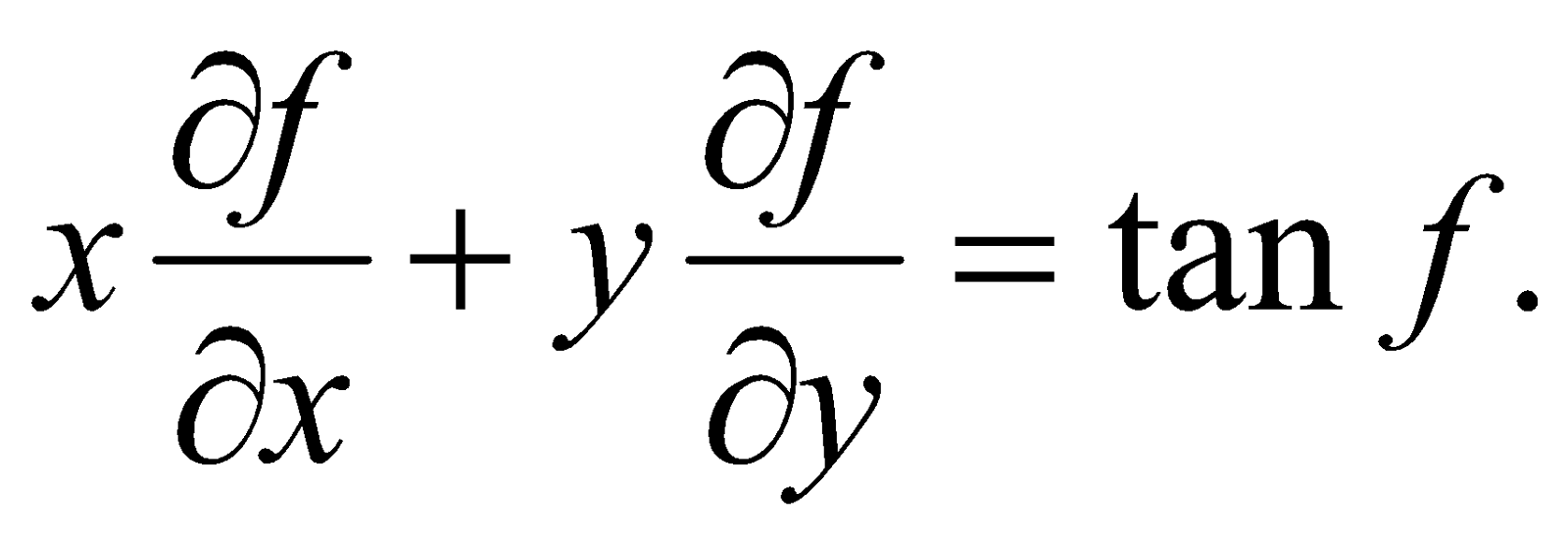3
Or
If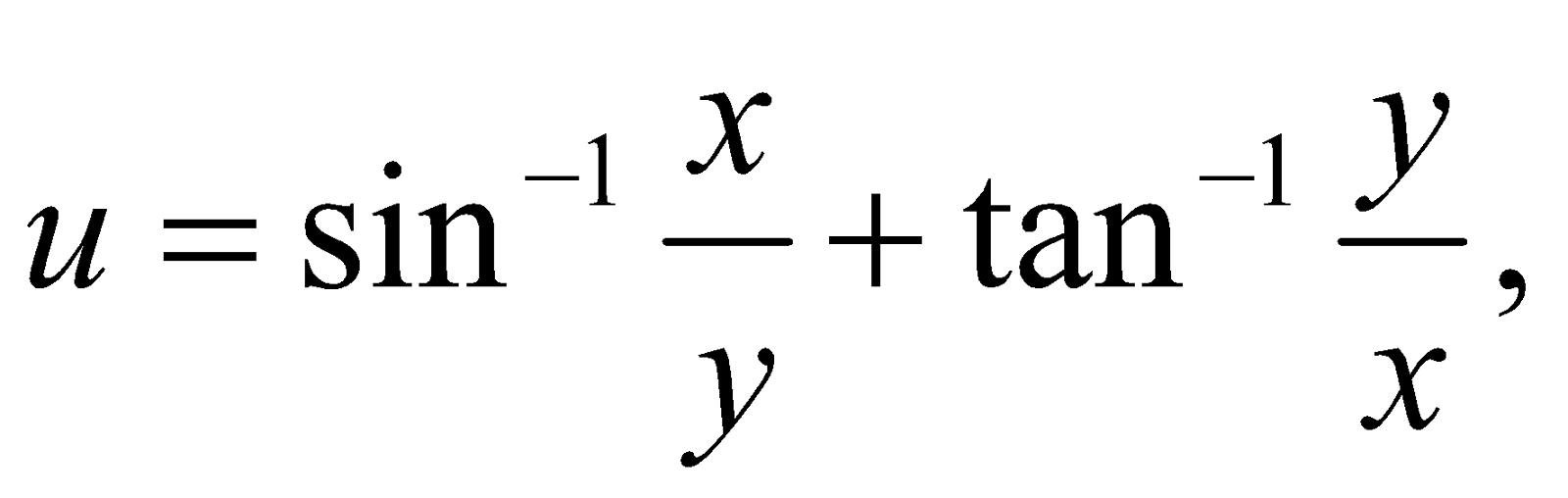then show that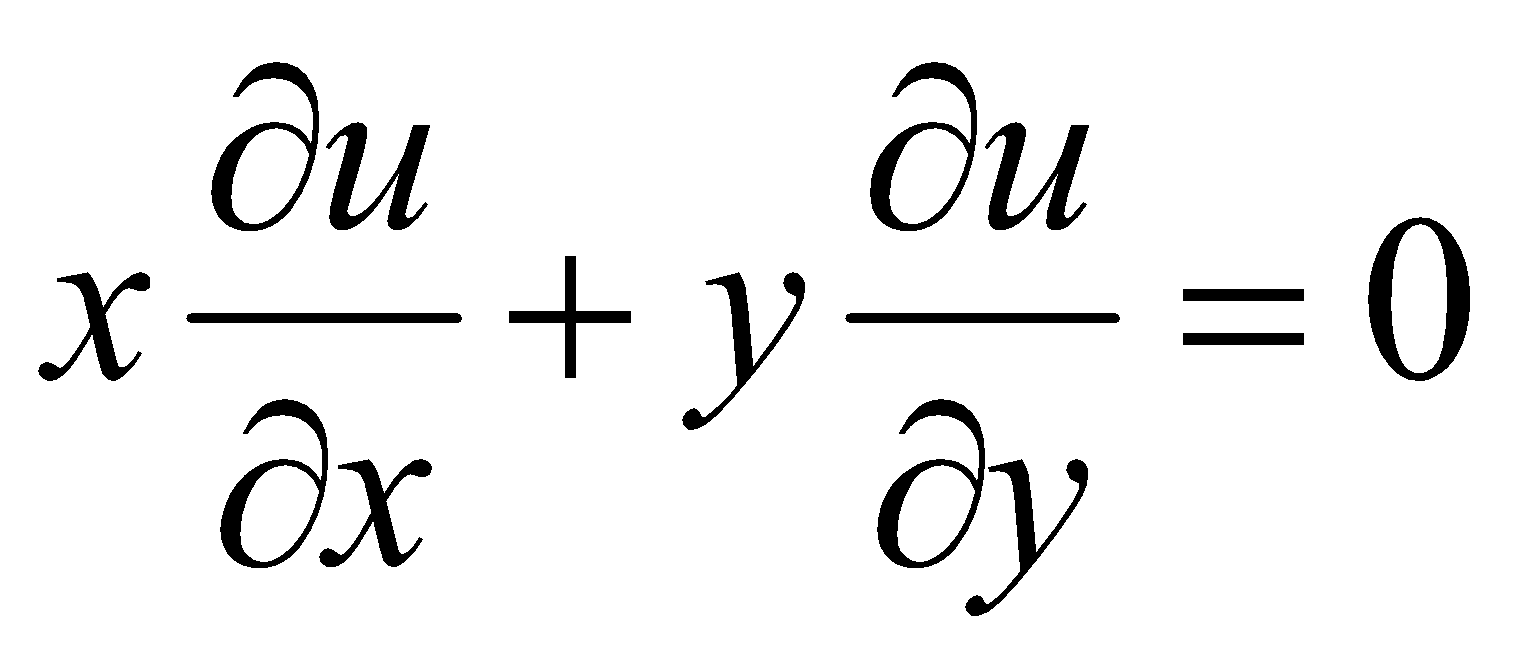9. (a) Write the condition when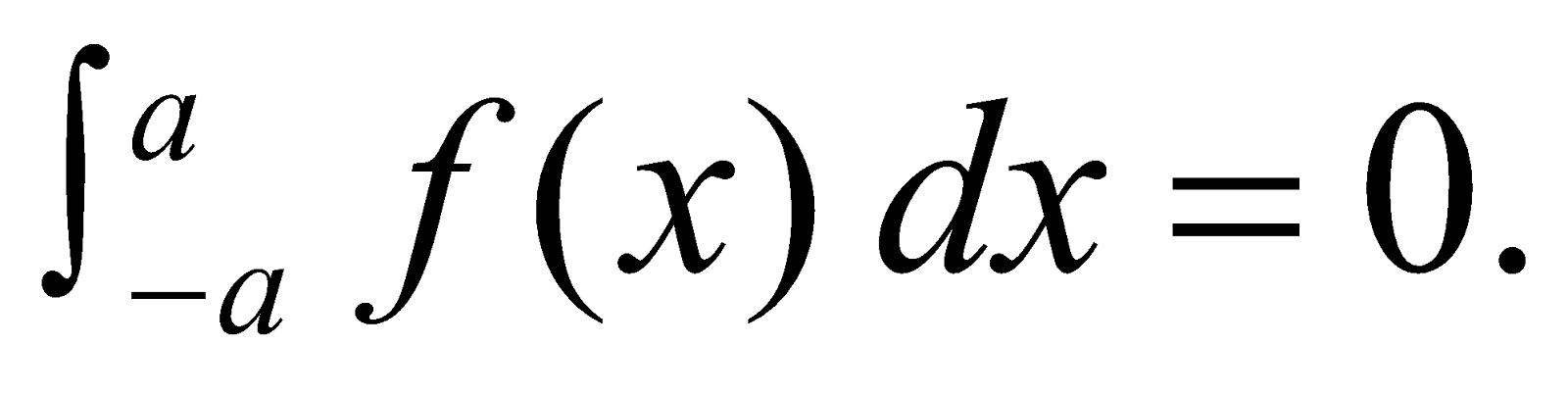1
(b) Evaluate: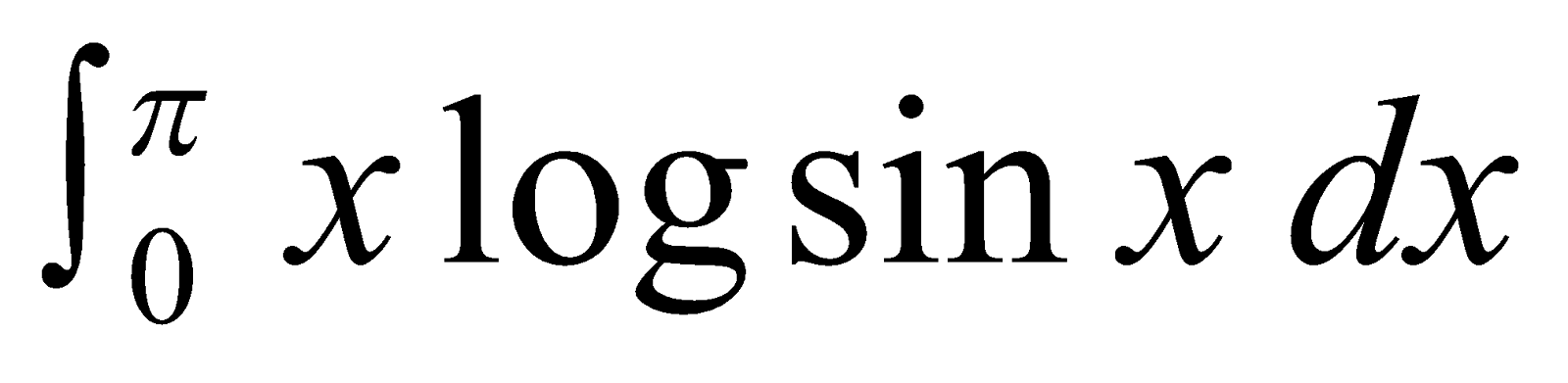5
(c) Evaluate: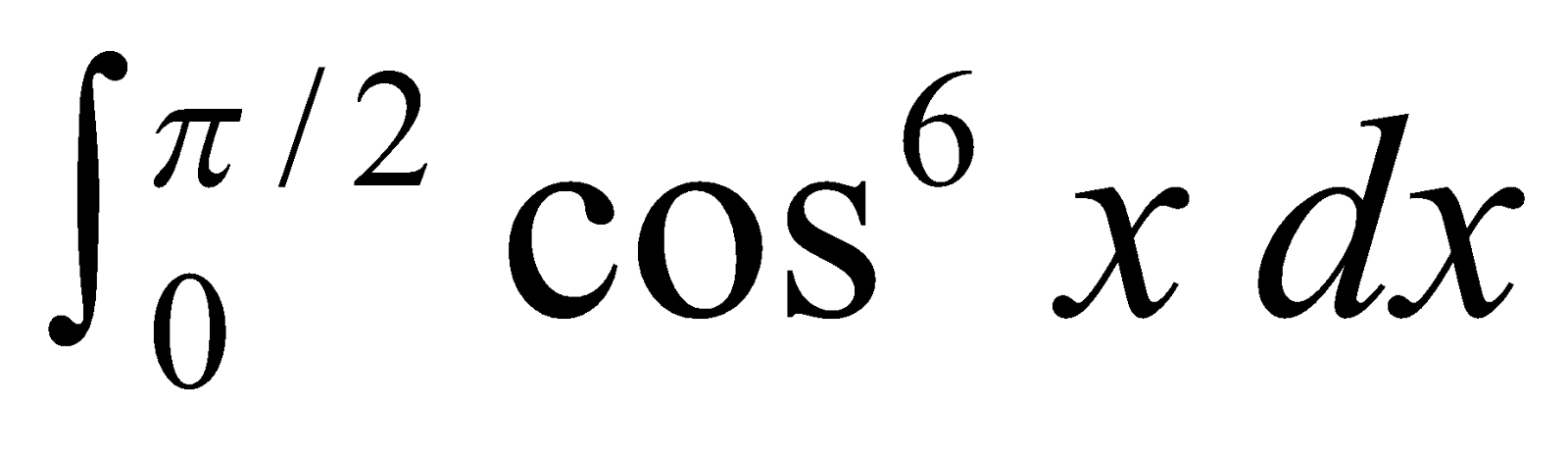4
Or
Find the length of the curve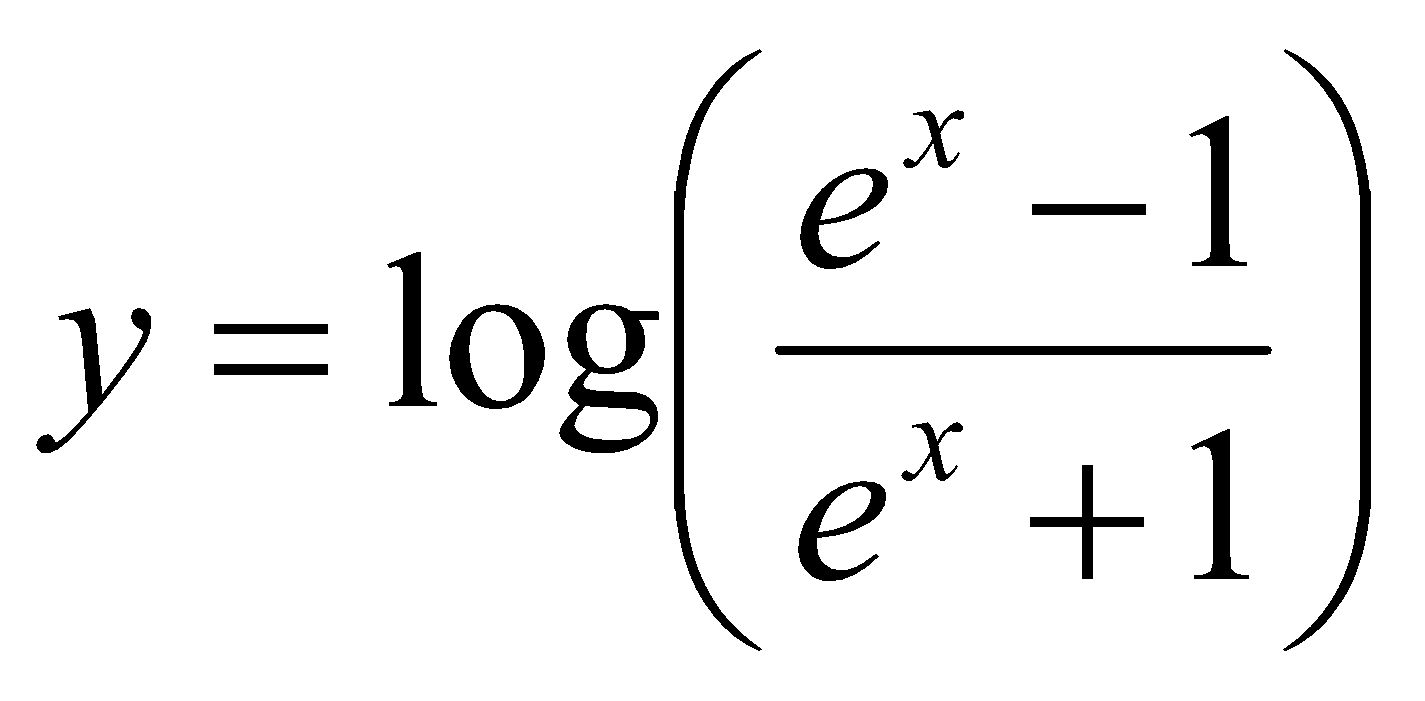from 1 to 2.

***